# Karnataka 2nd PUC Board (KSEEB) Maths Class 12 Question Paper 2019

Karnataka 2nd PUC board Maths 2019 (Class 12) Question paper with solutions are provided here in a downloadable pdf format and also in the text, so that the students are aware of the exact difficulty level of the question papers. Along with the solutions, 2nd PUC maths question paper 2019 is also attached here for reference. Students can access all the Karnataka 2nd PUC board previous year maths question papers.

The solutions provided by BYJU’S will motivate the students to solve questions from different concepts and aim to score high marks in the upcoming KSEEB board examination. Solving 2019 Maths question papers helps the students to understand the essential topics, types of questions asked, time management, marking scheme. It also helps in improving speed and efficiency.

### KSEEB 2nd PUC (Class 12th) Maths Question Paper With Solutions 2019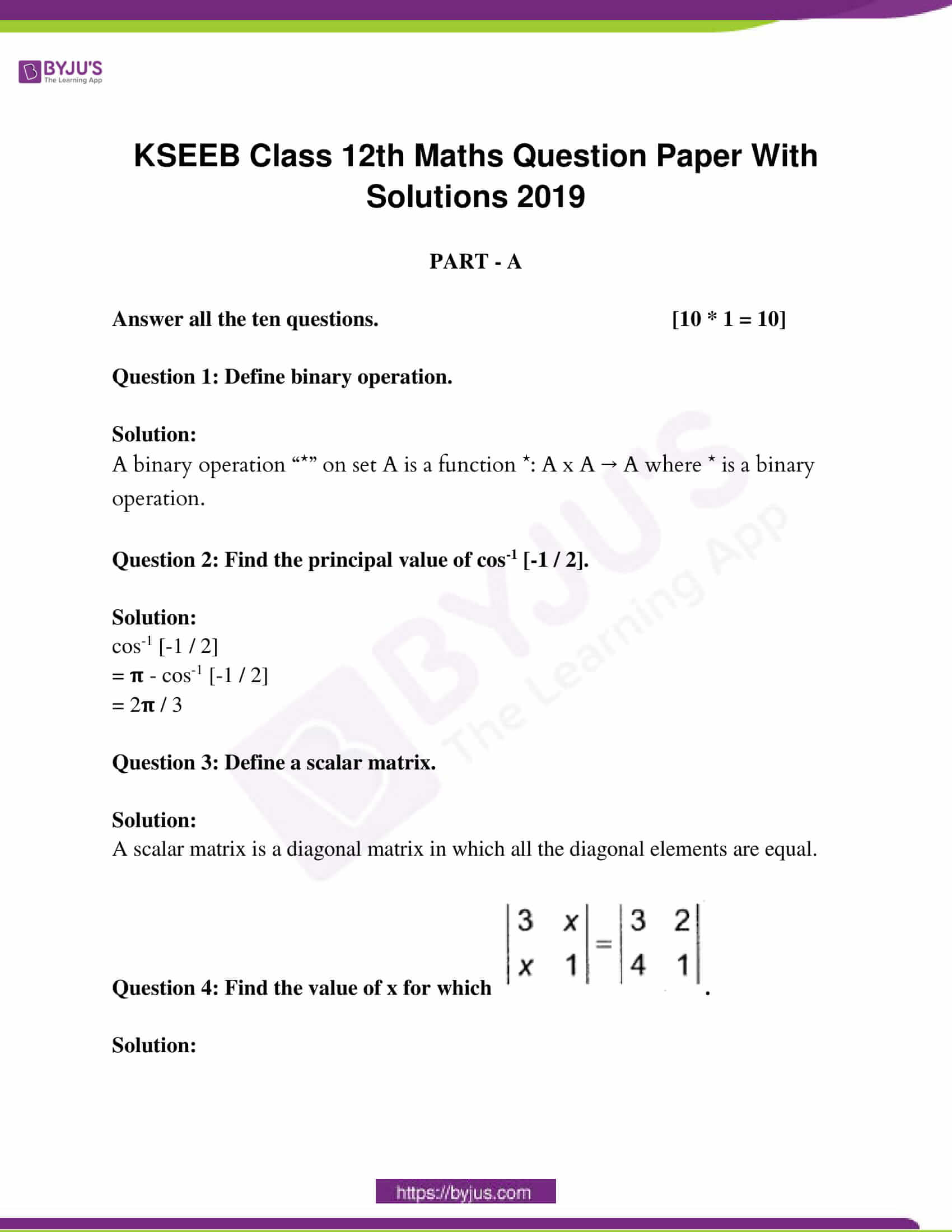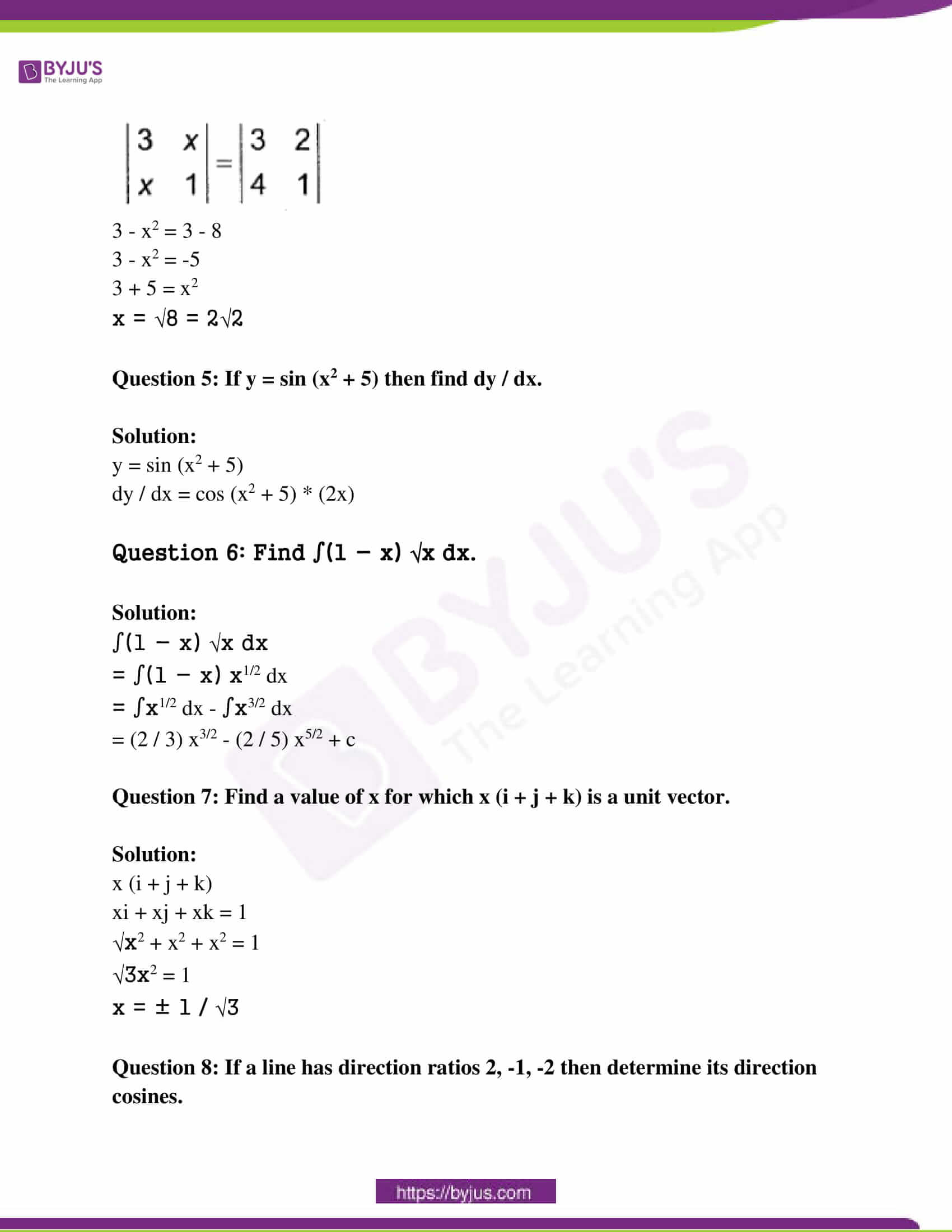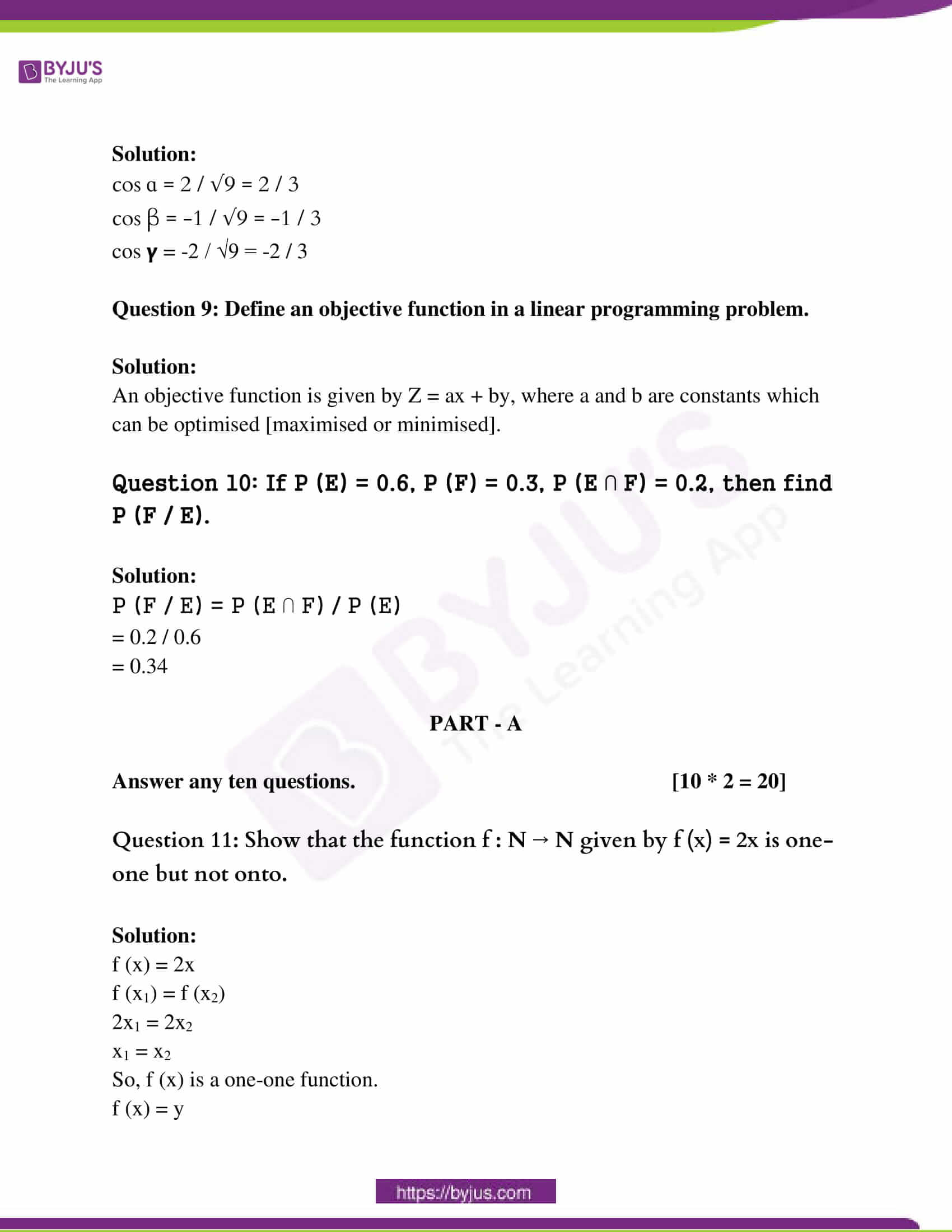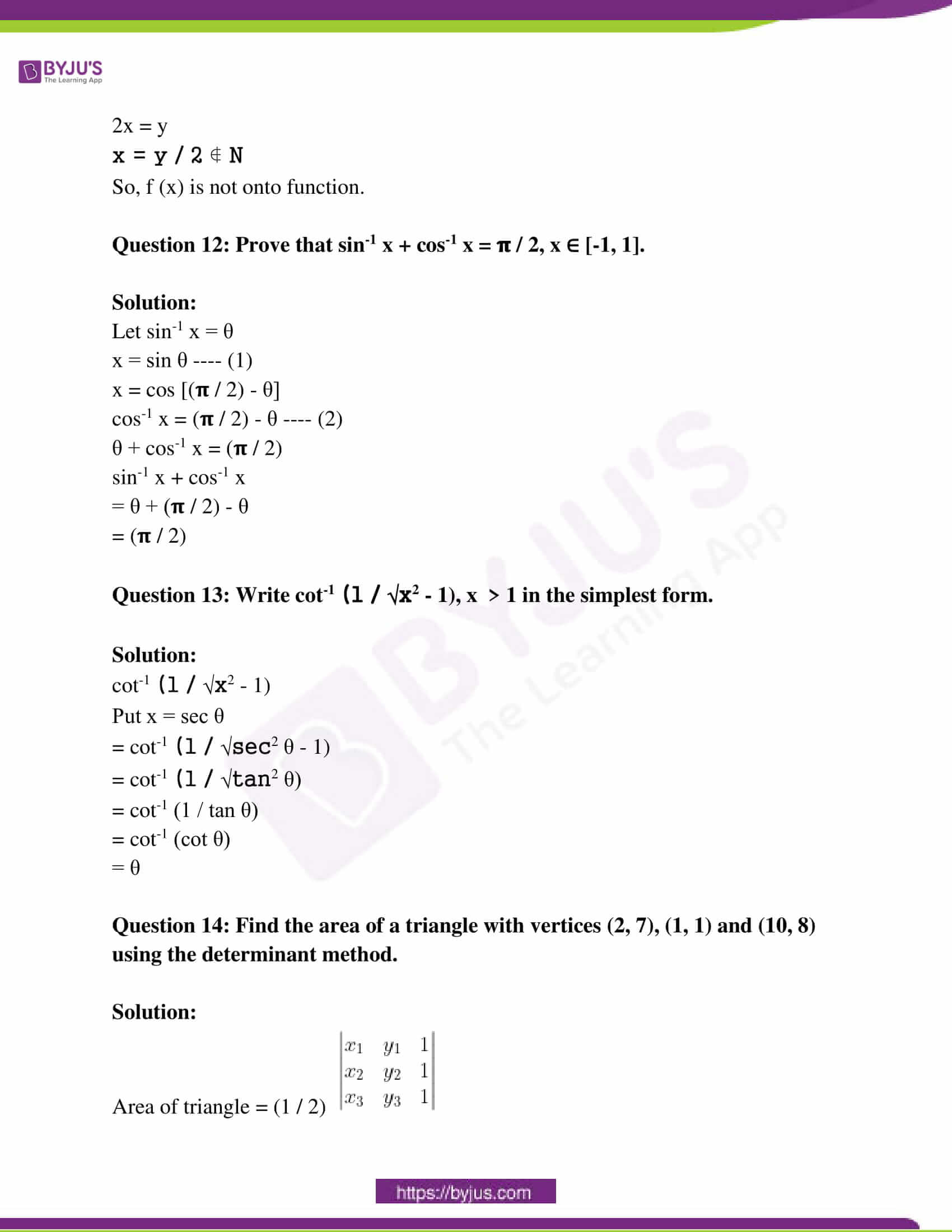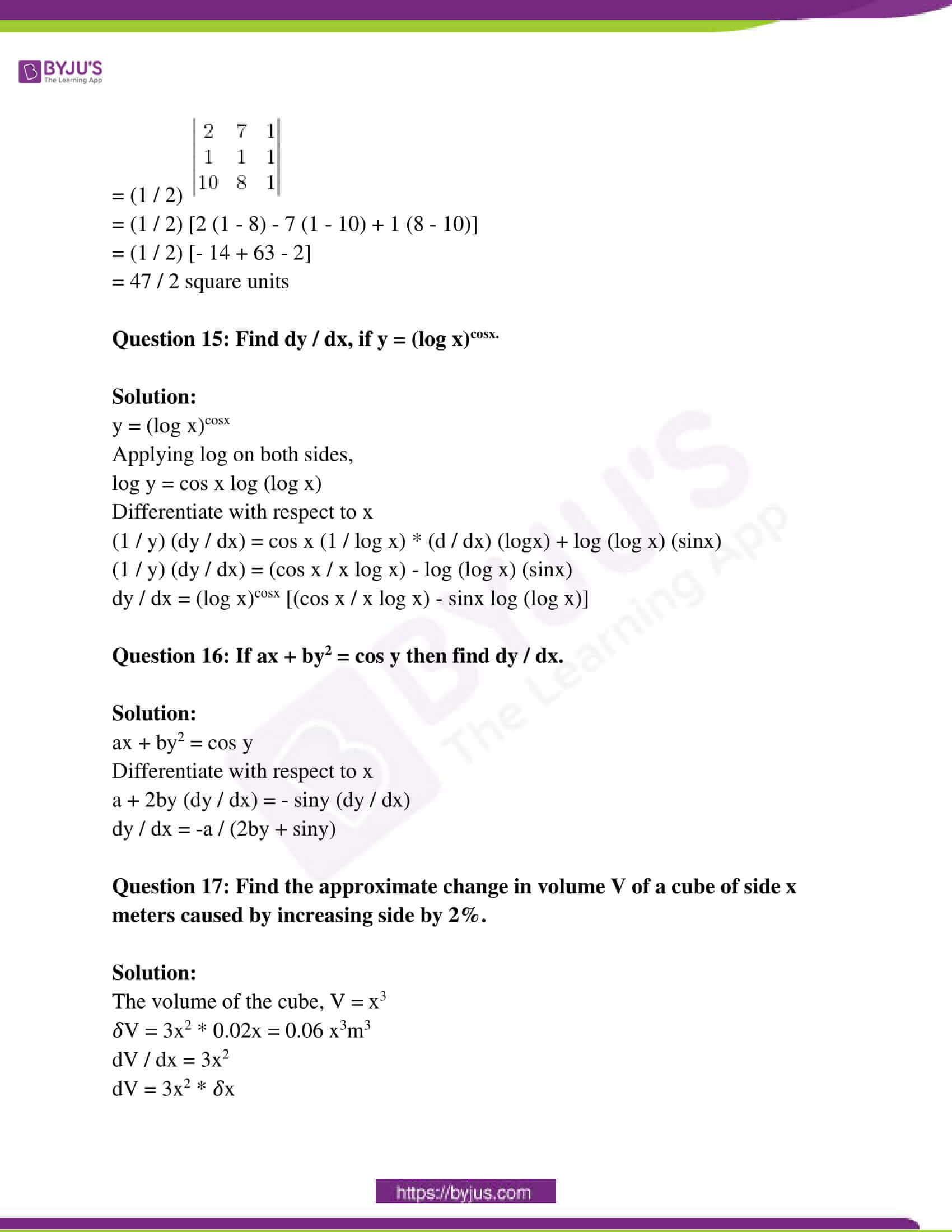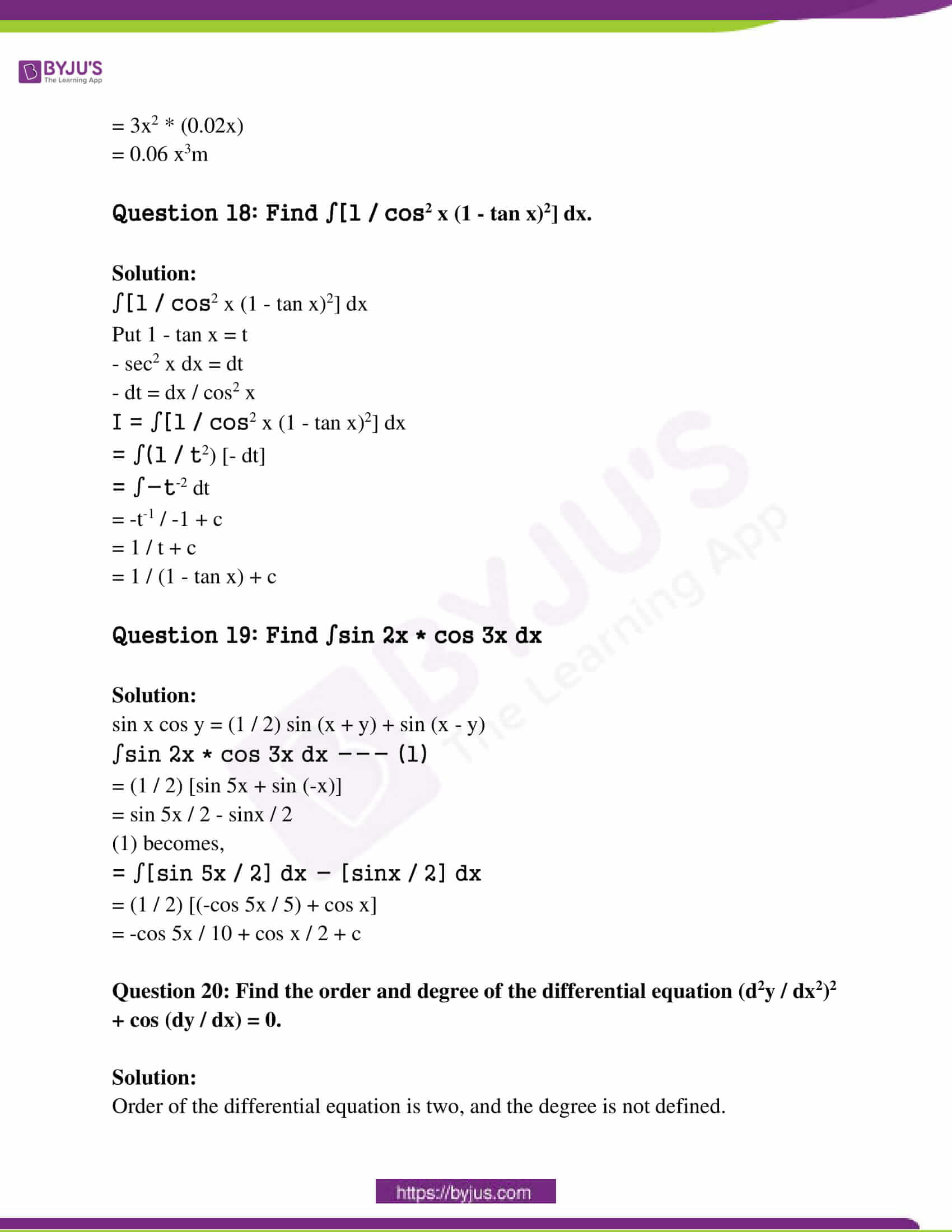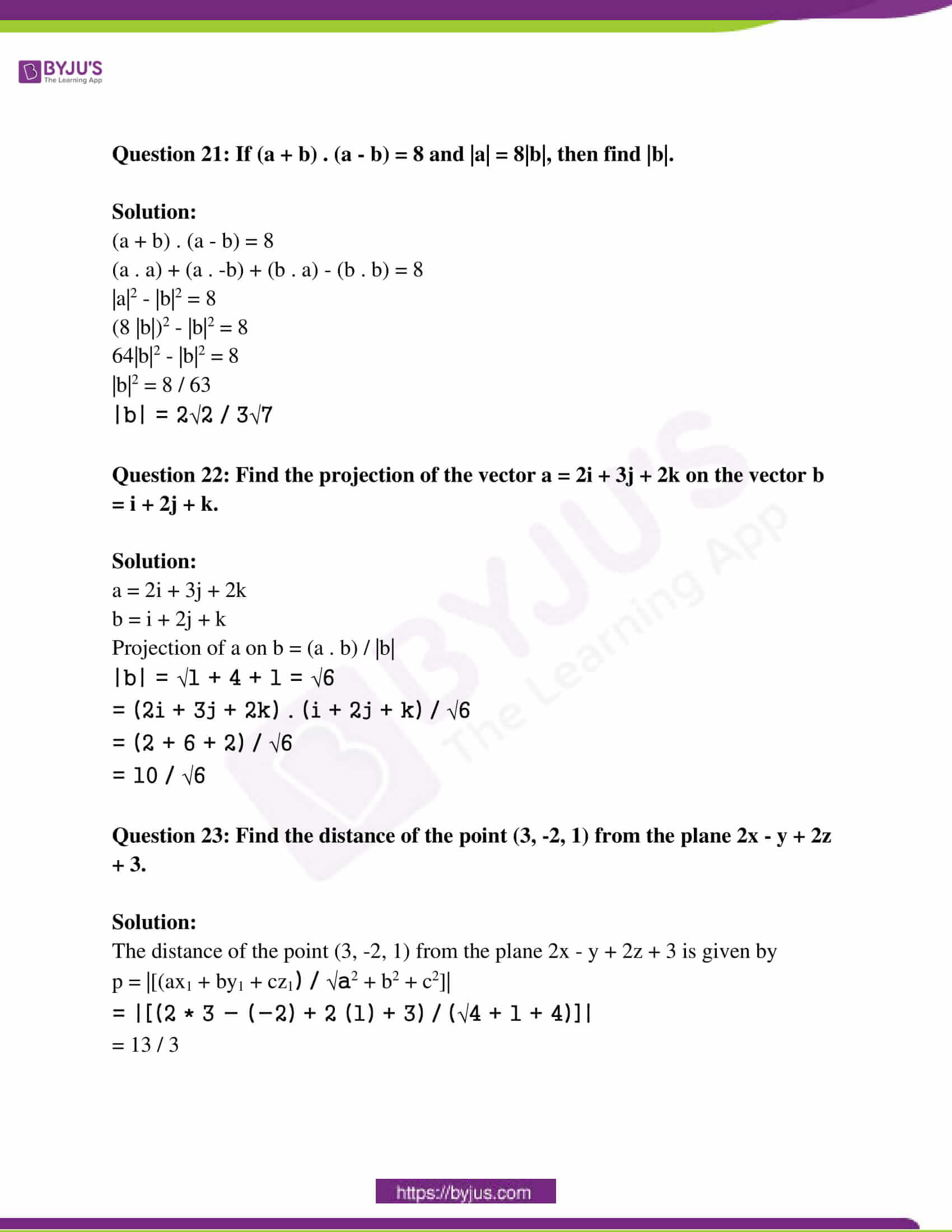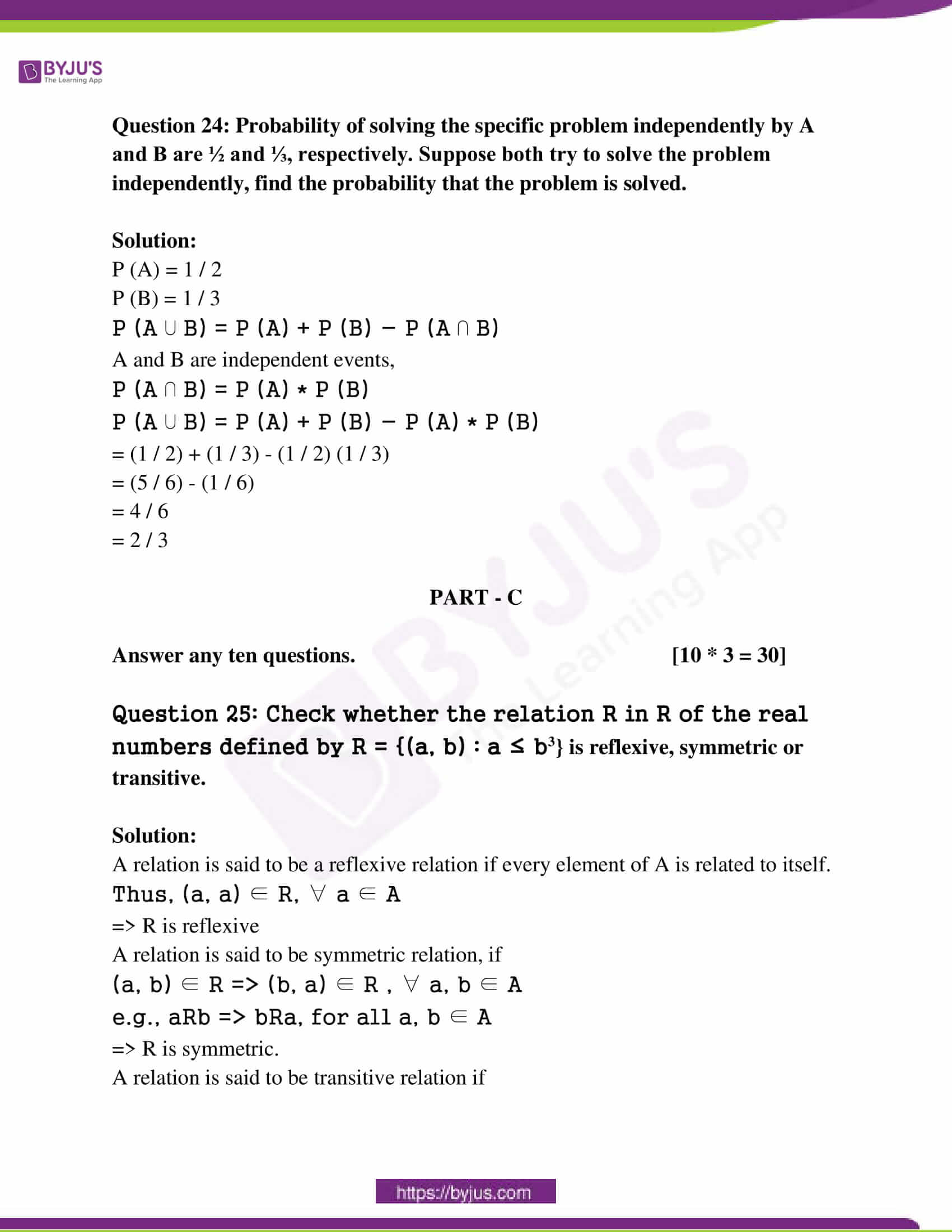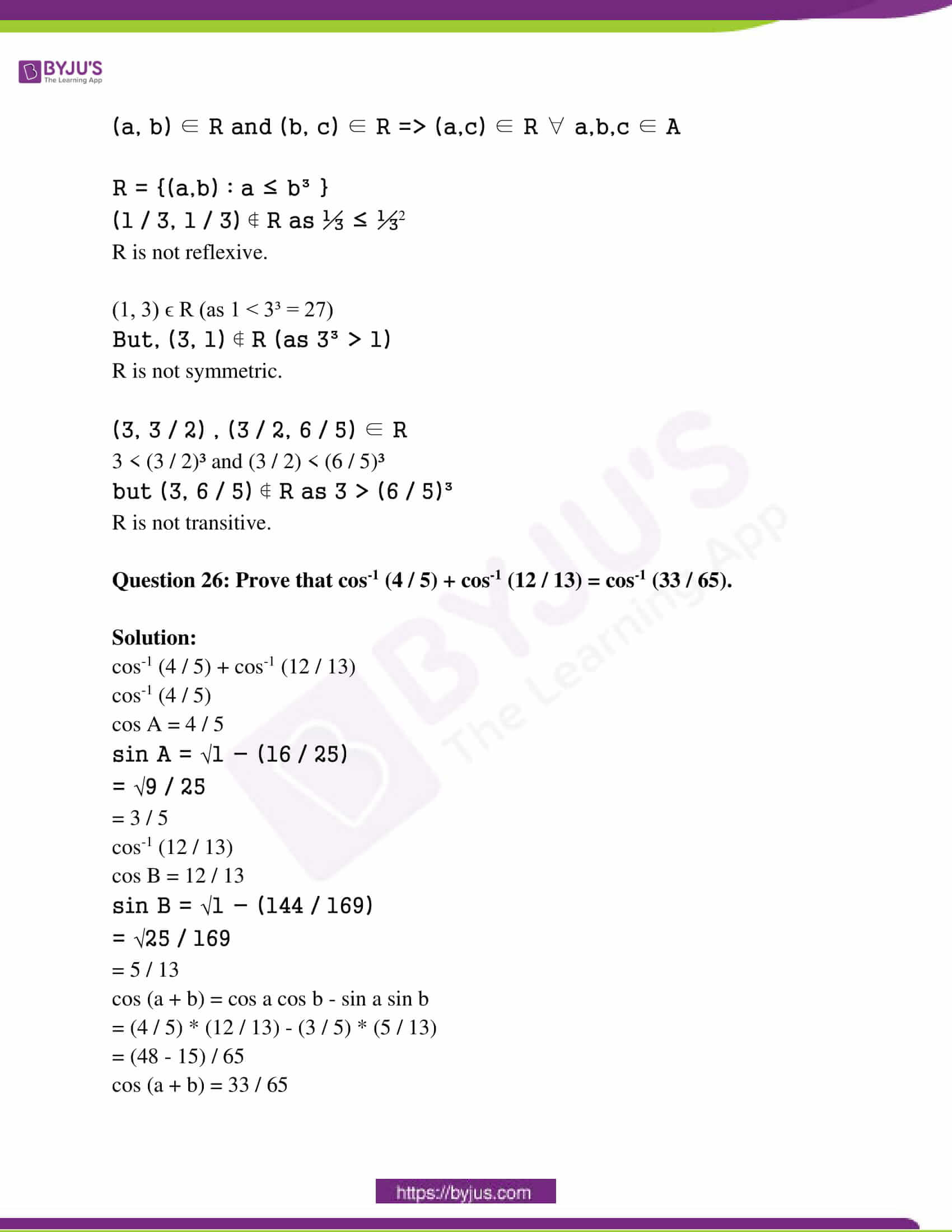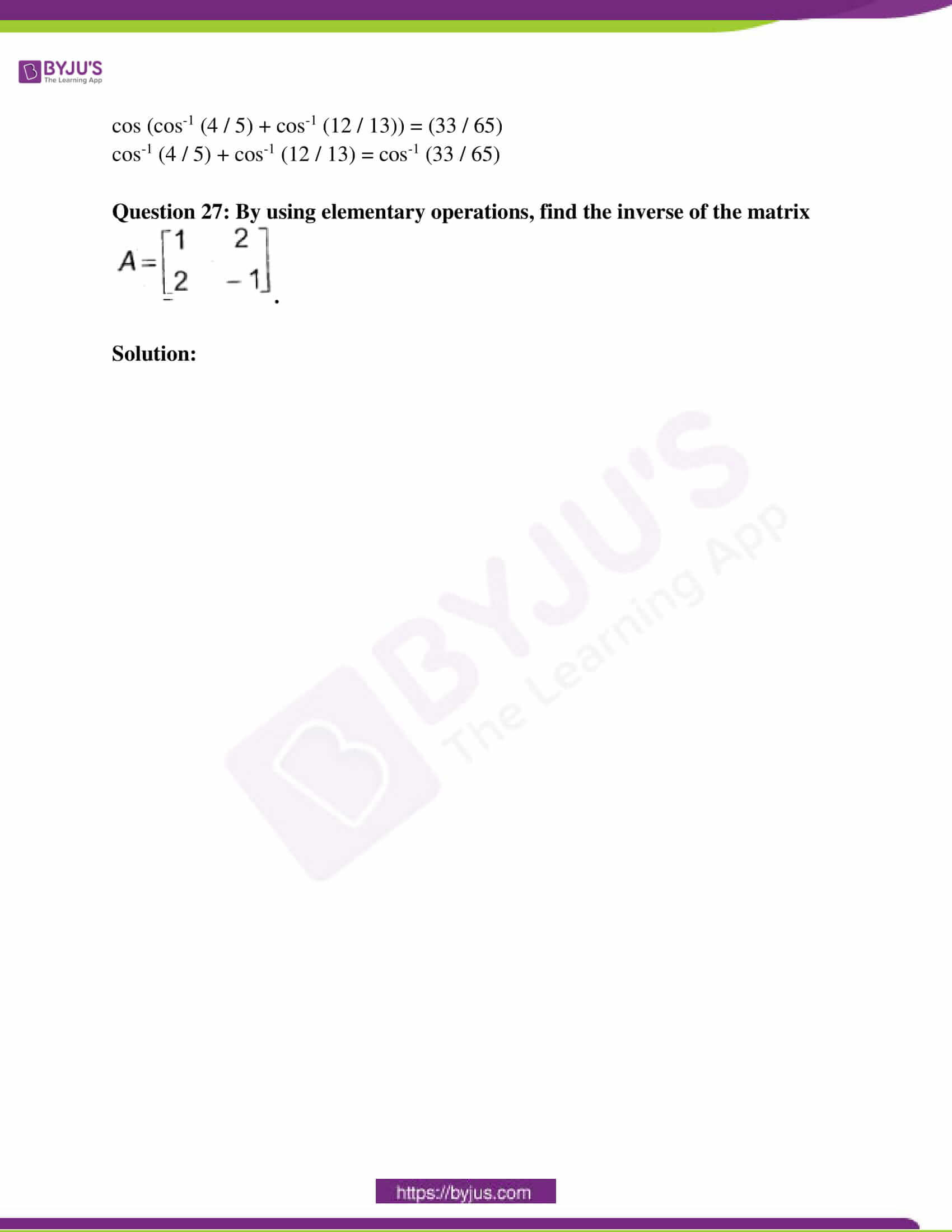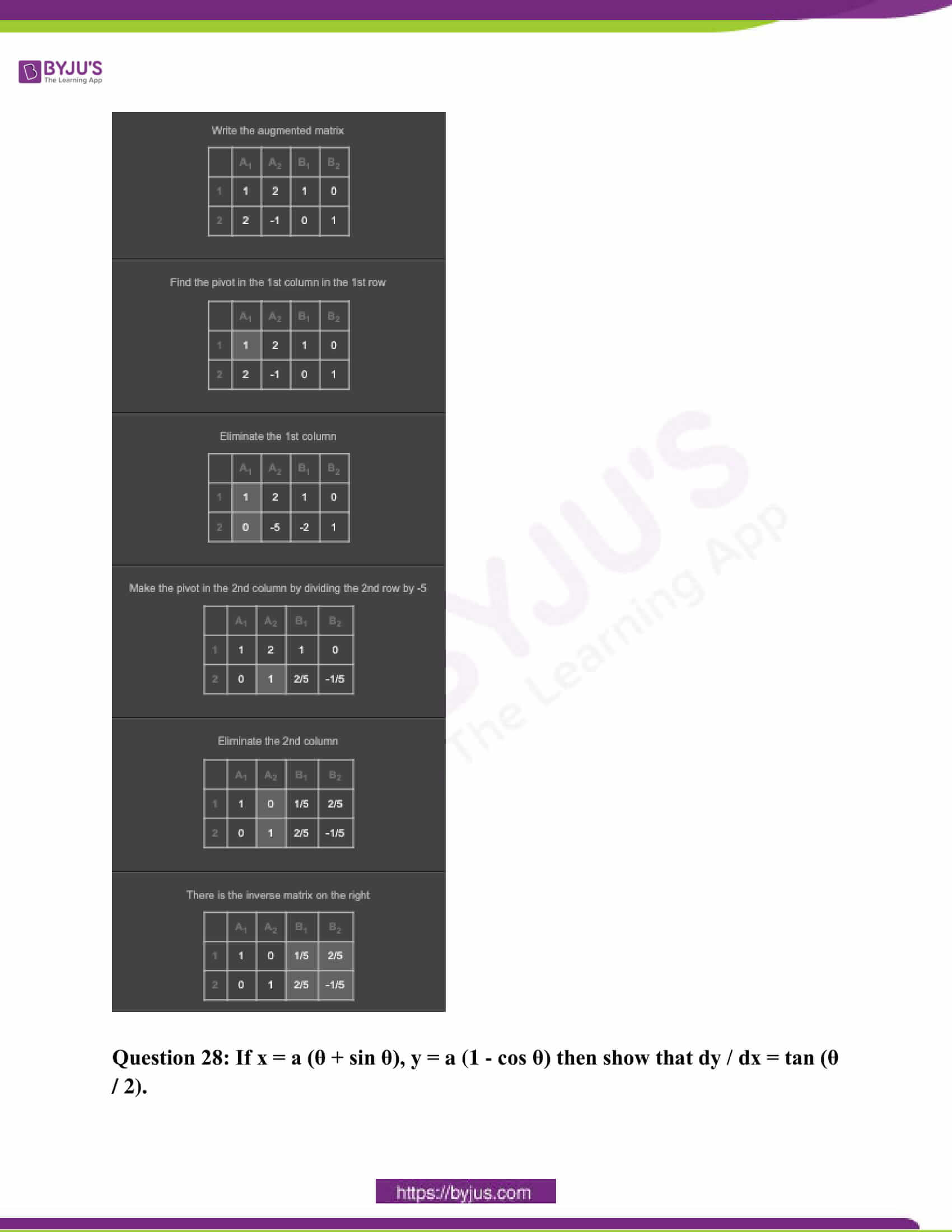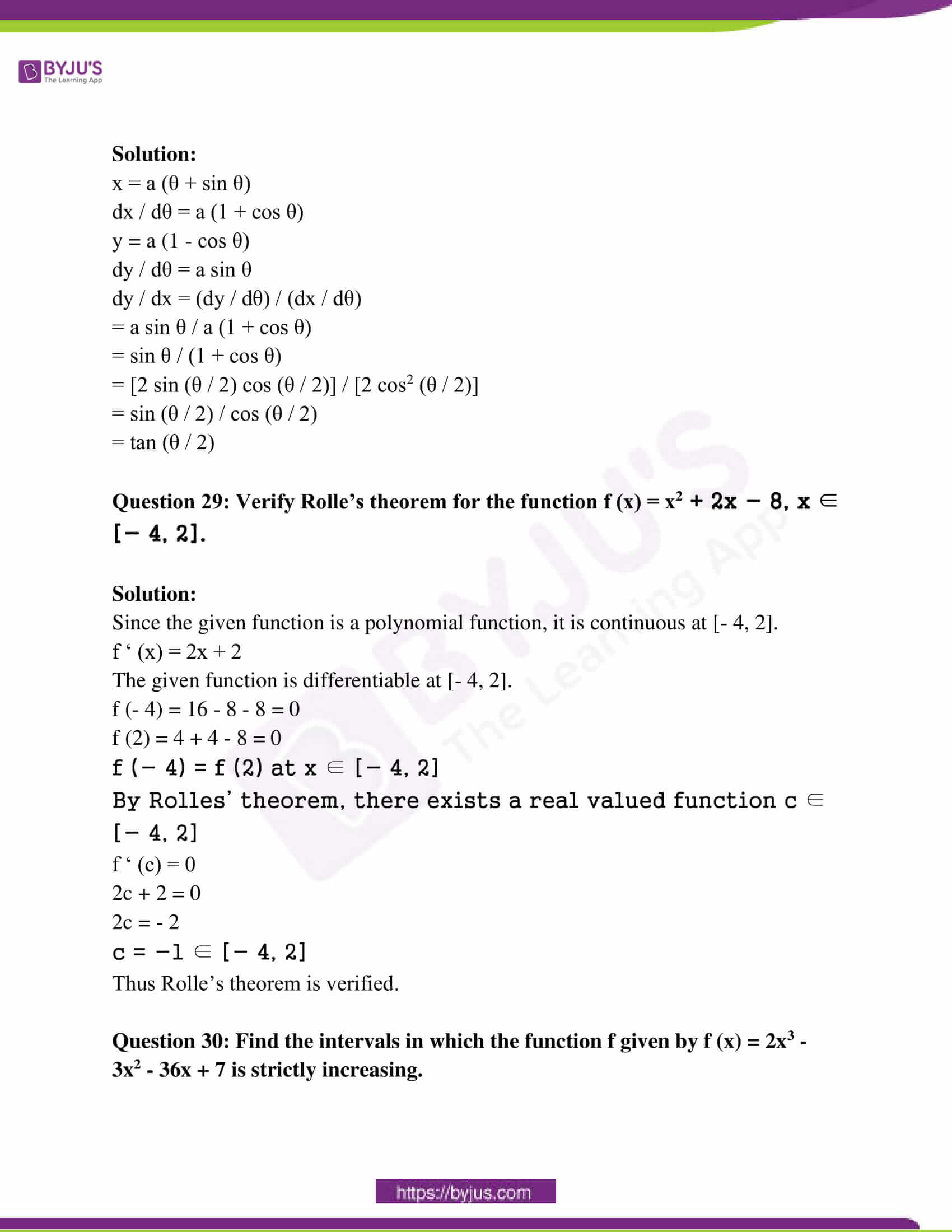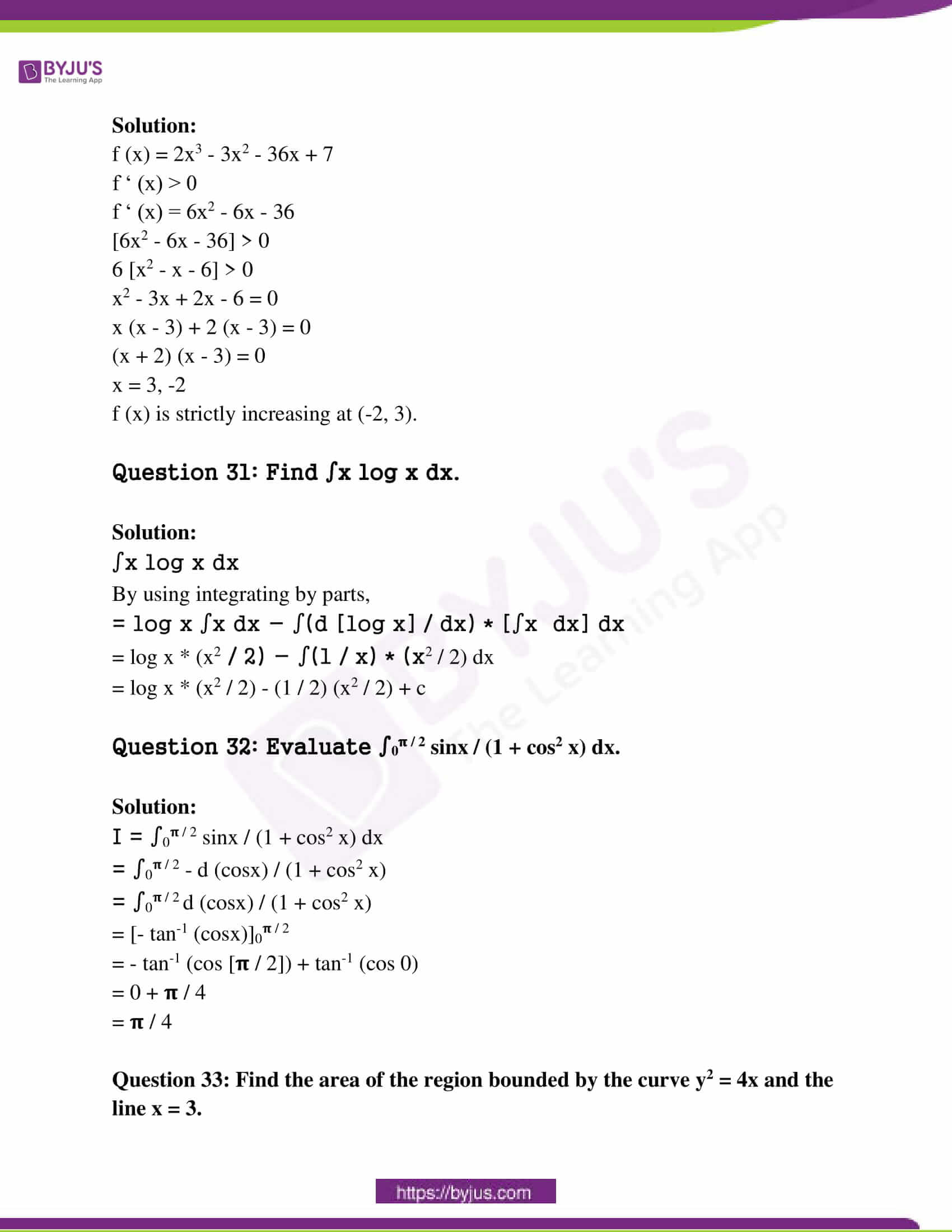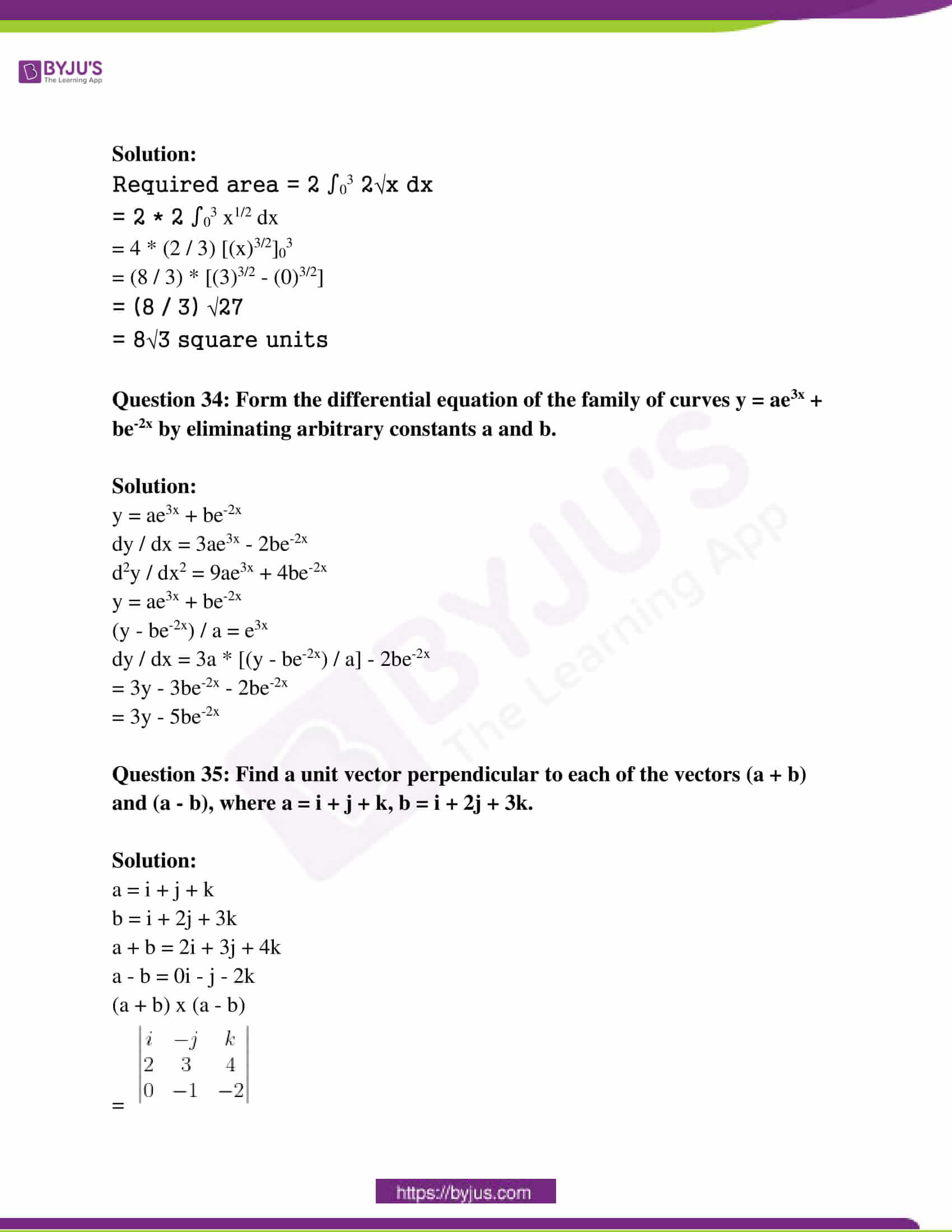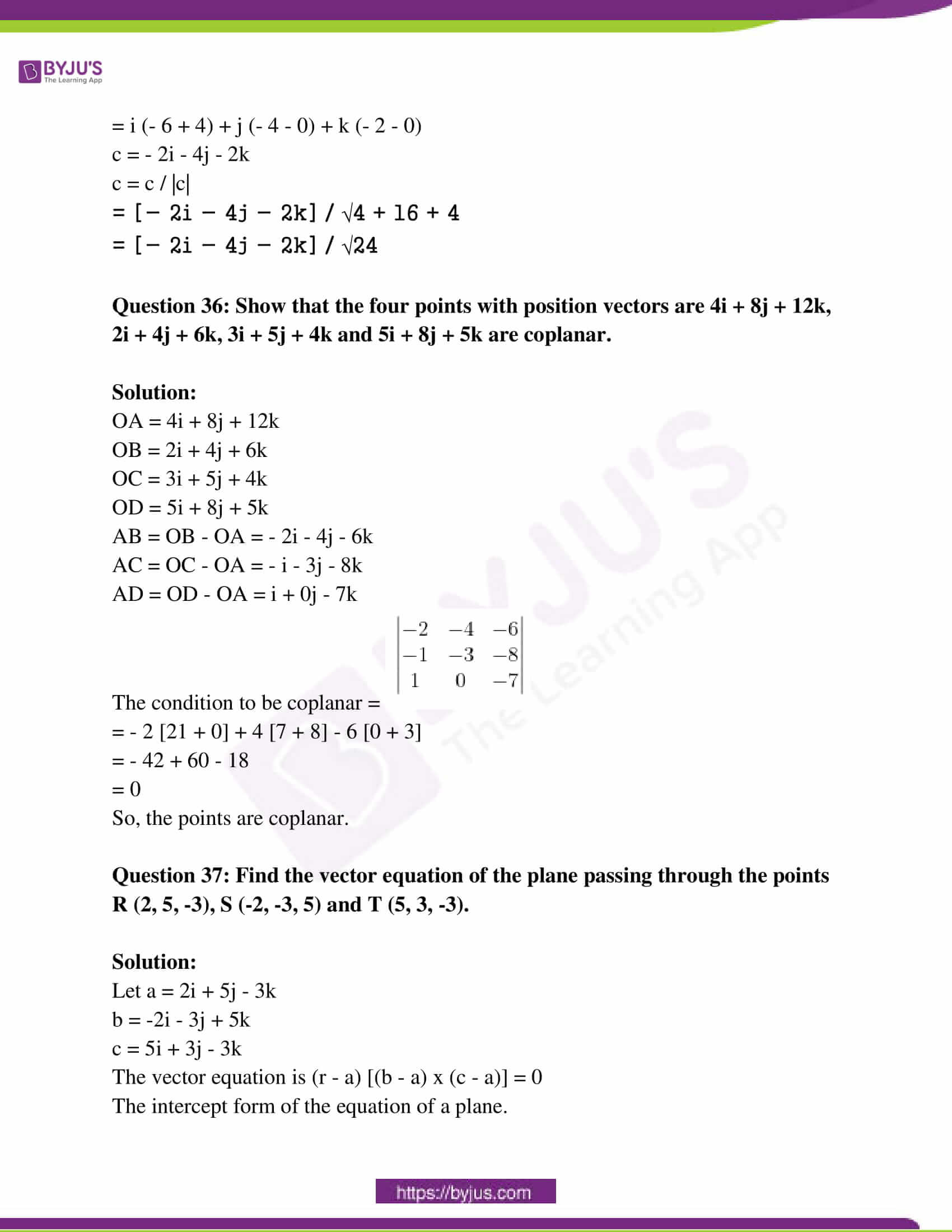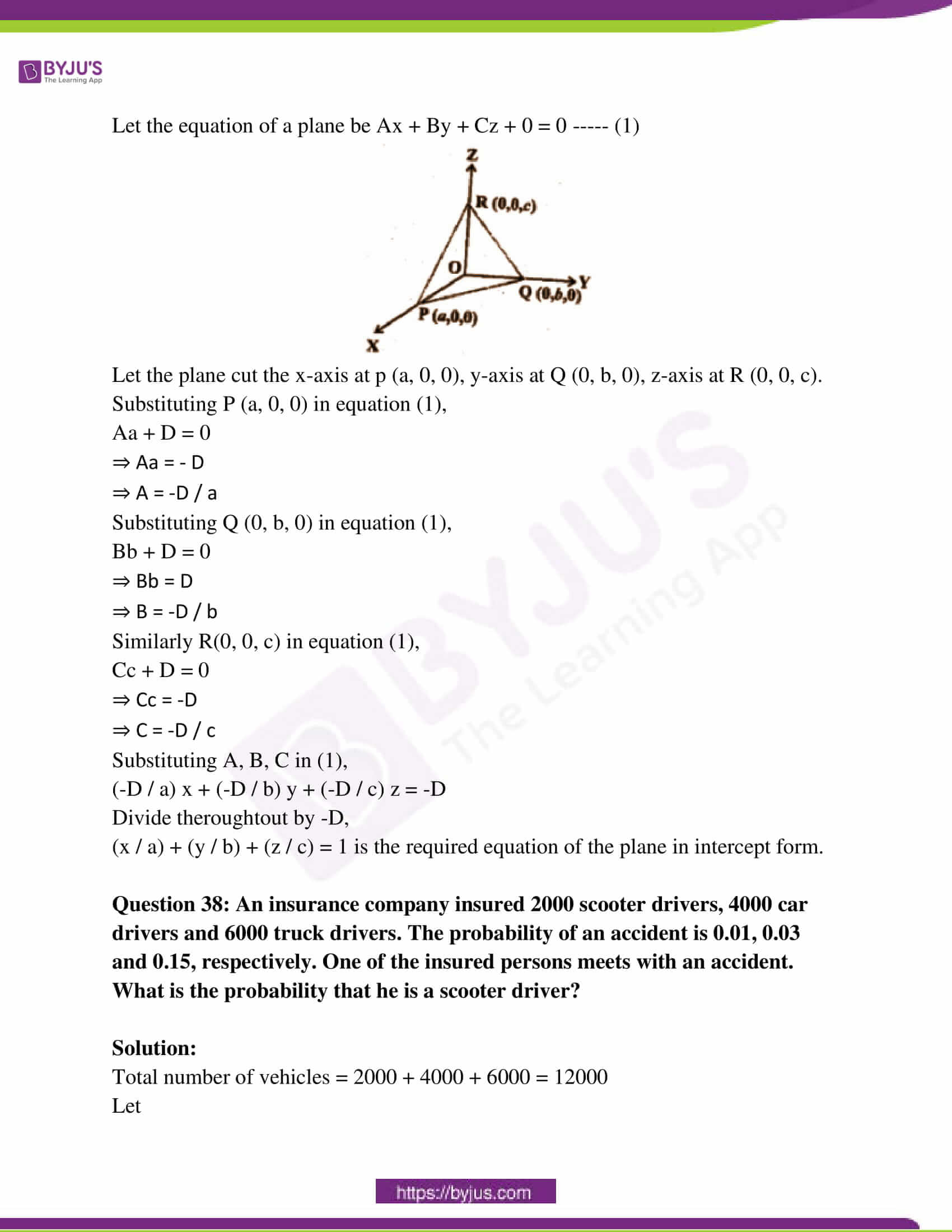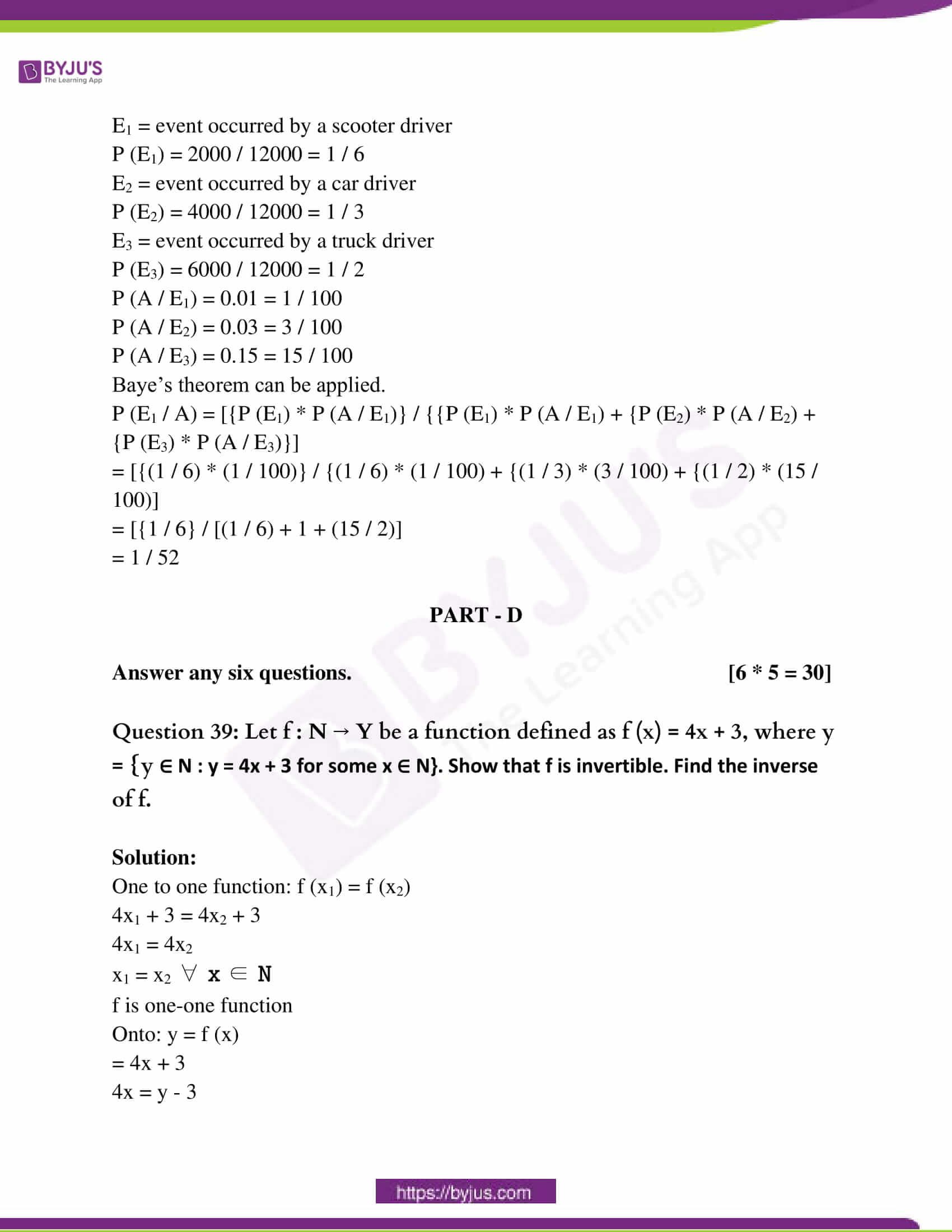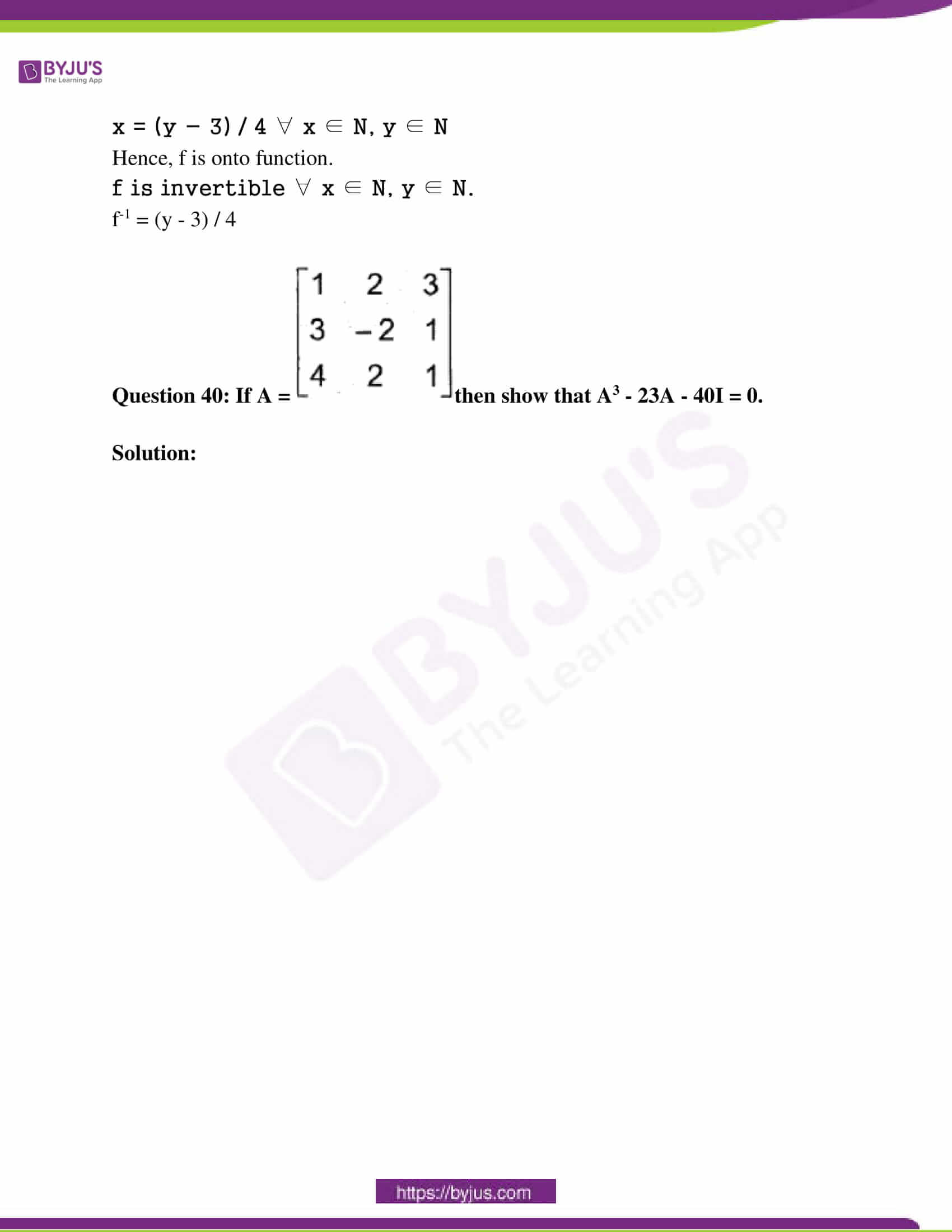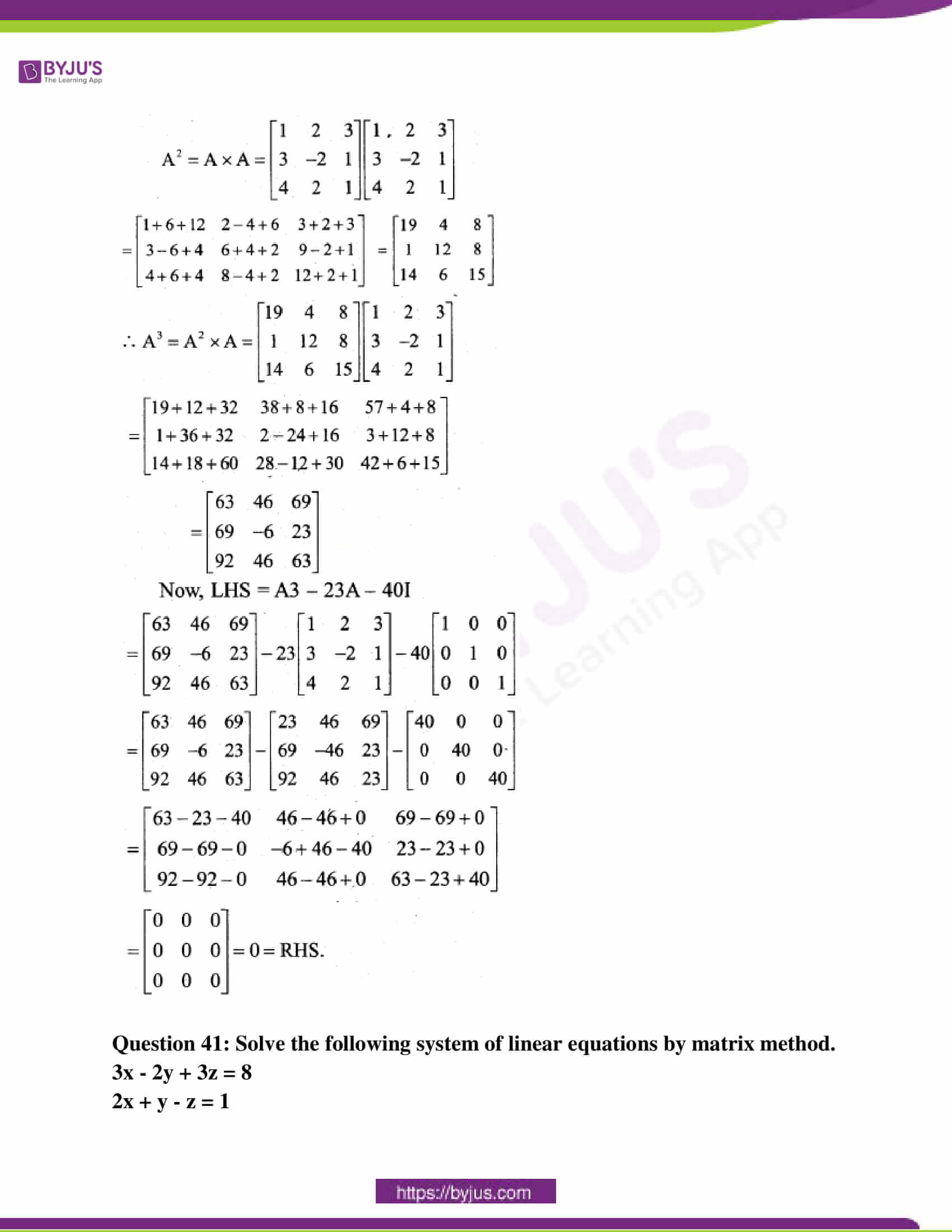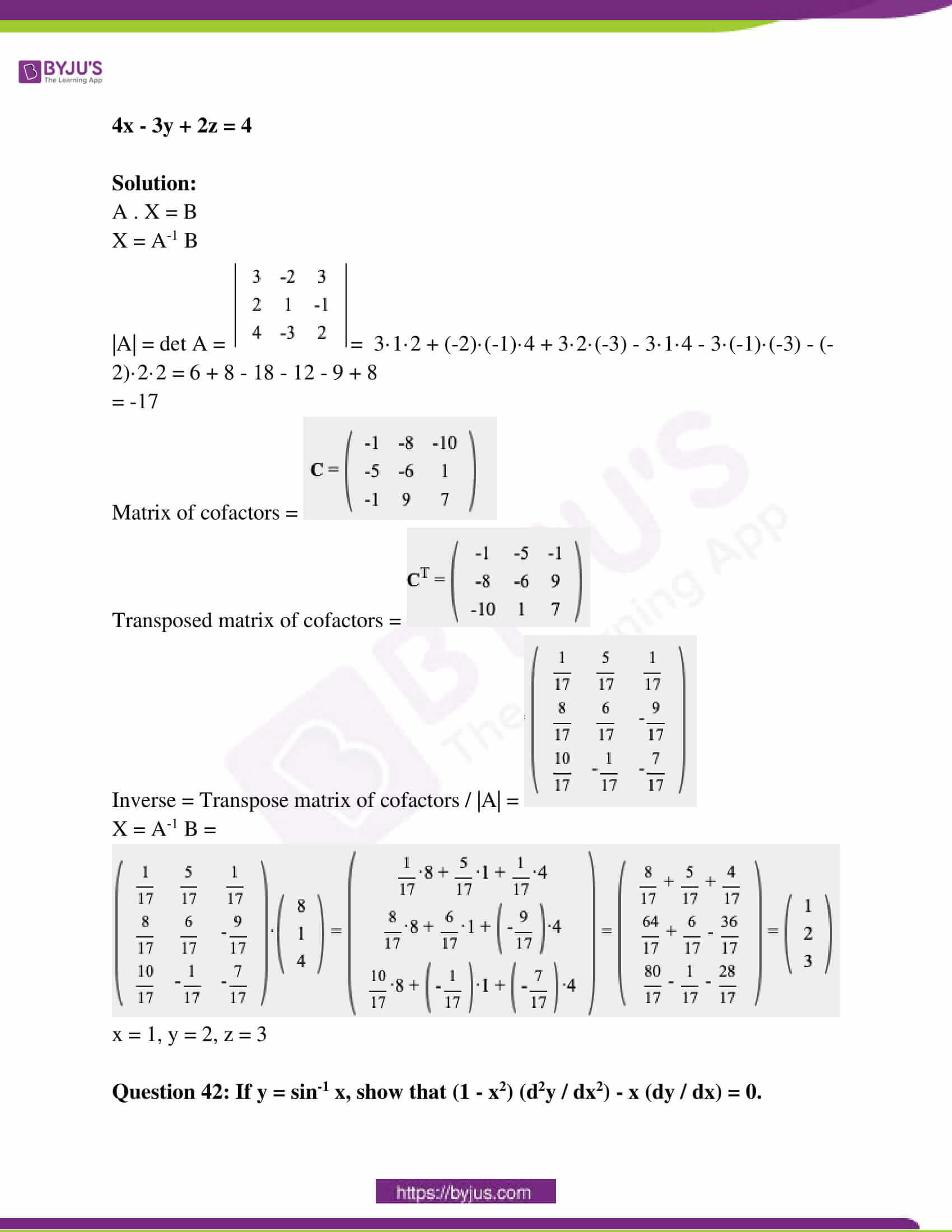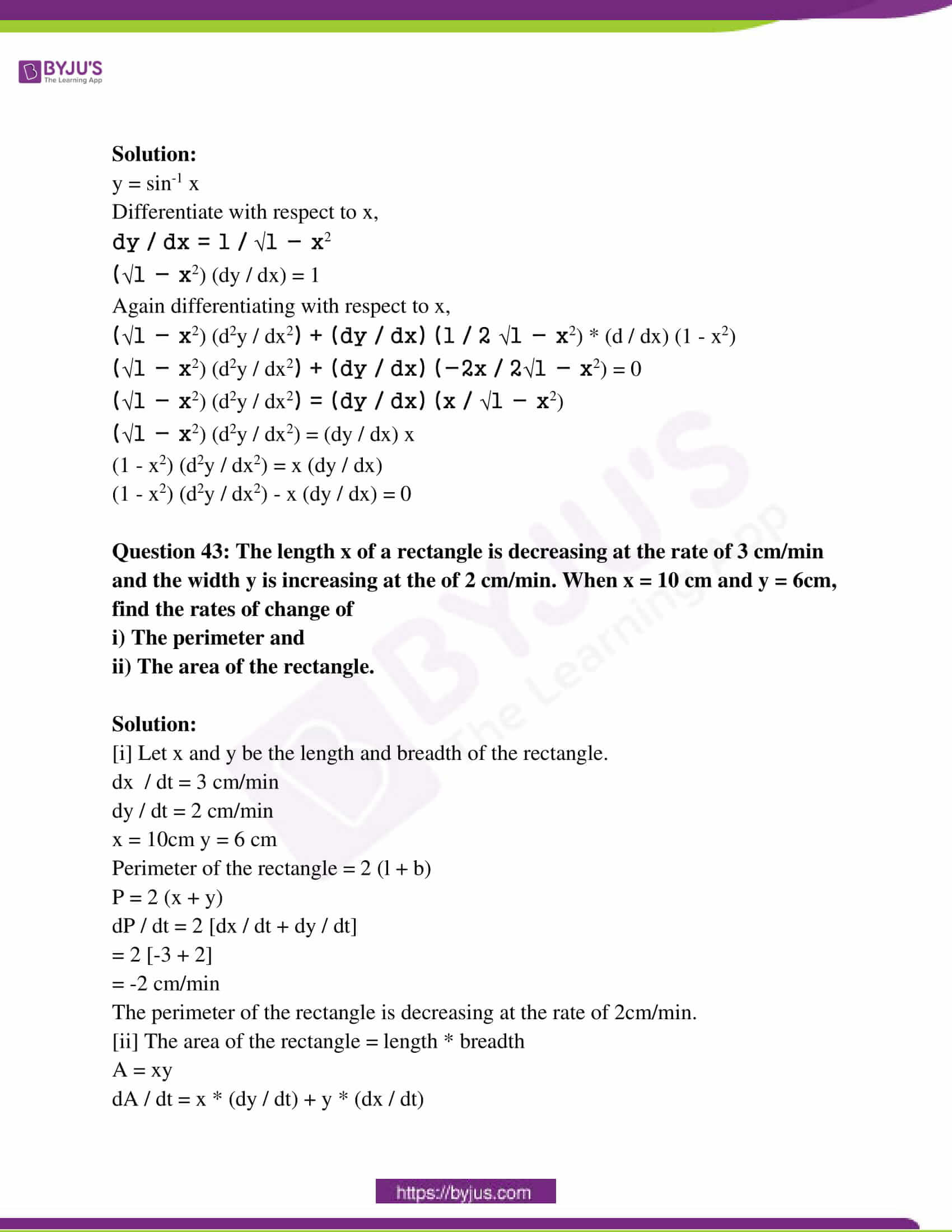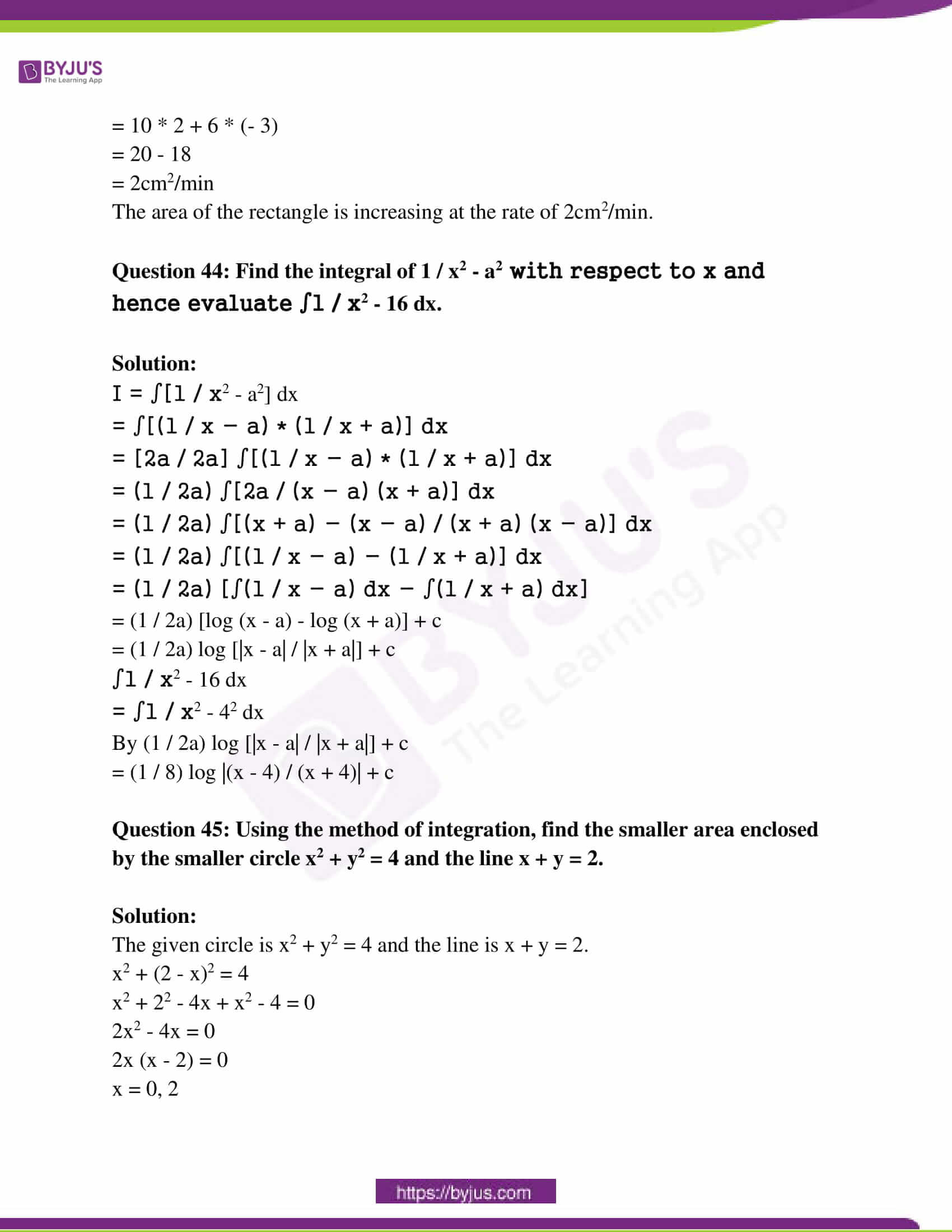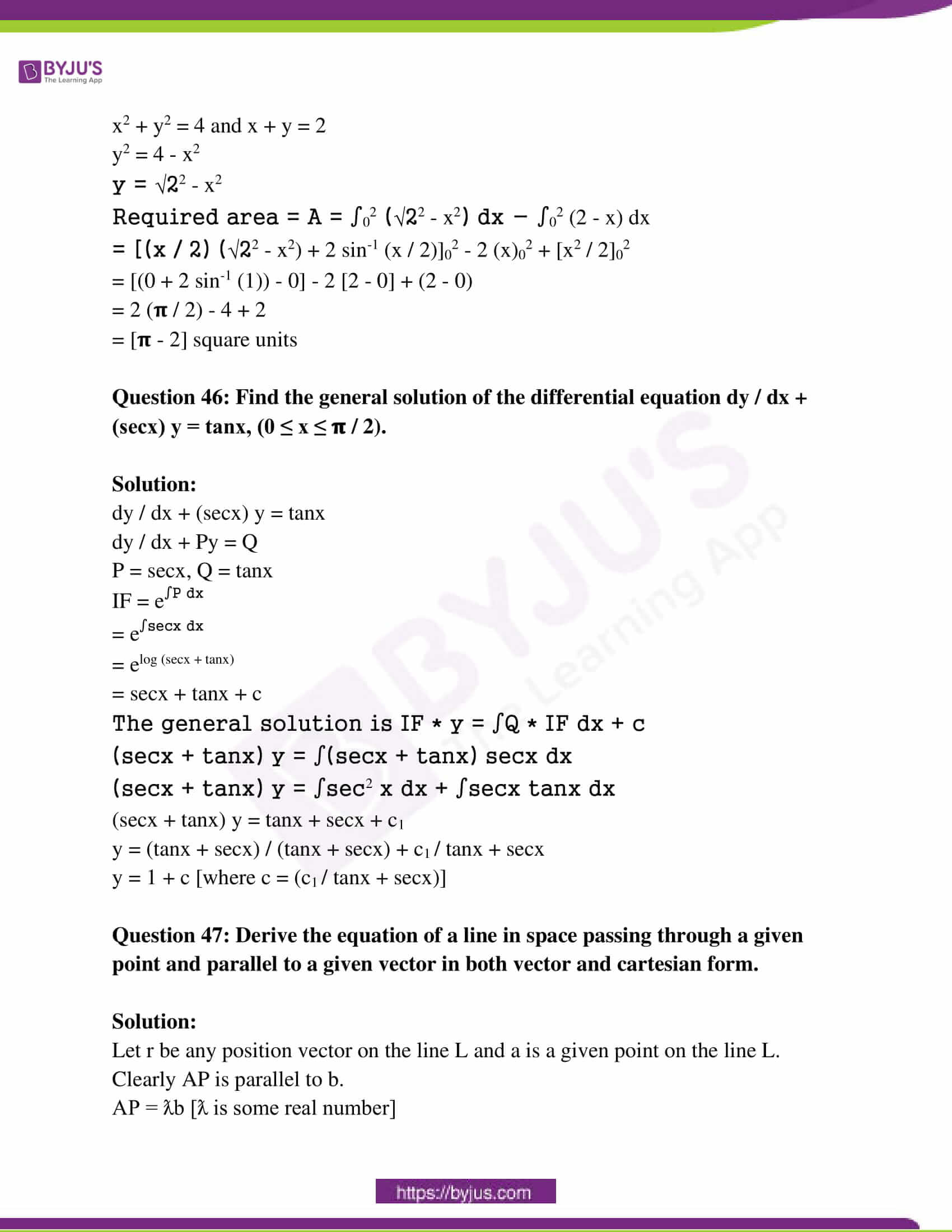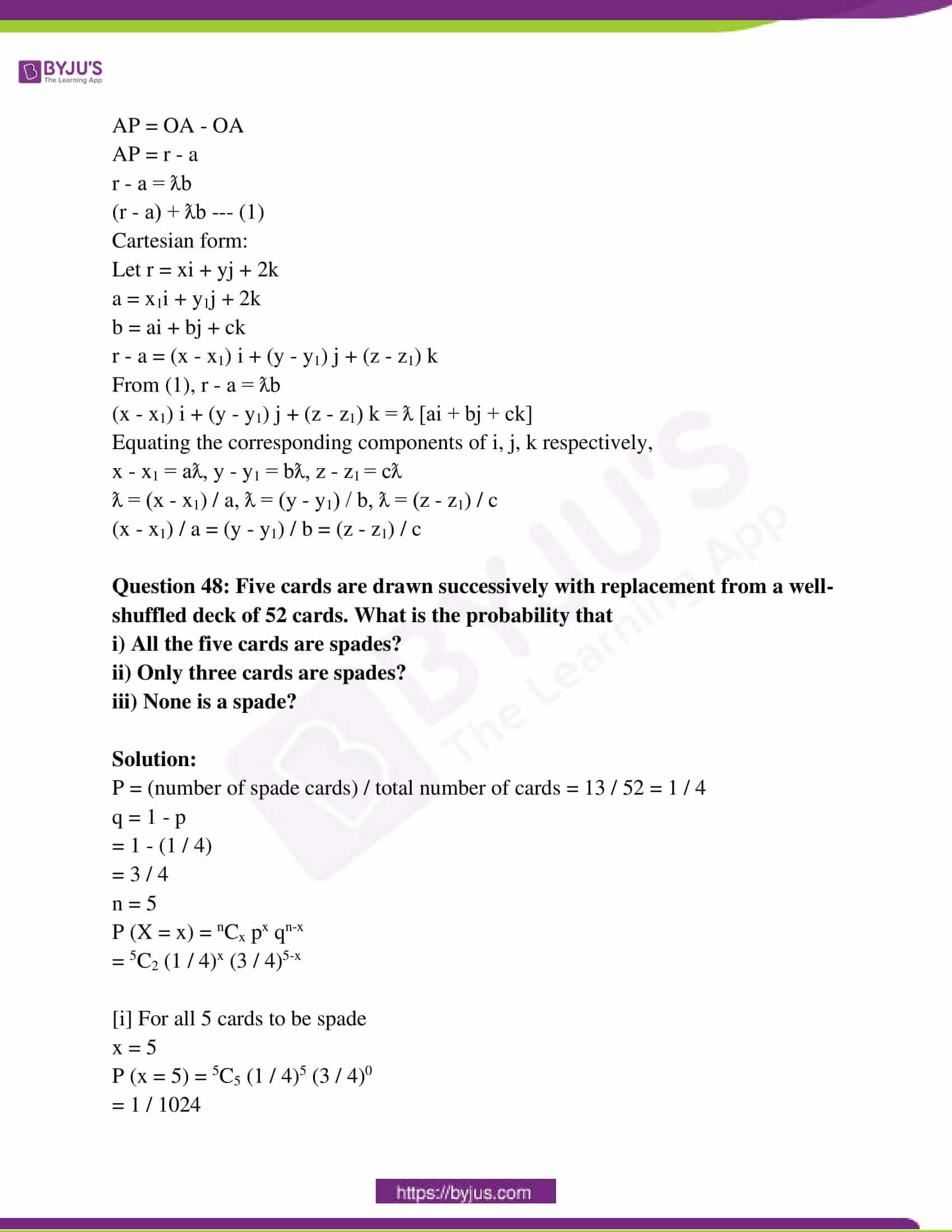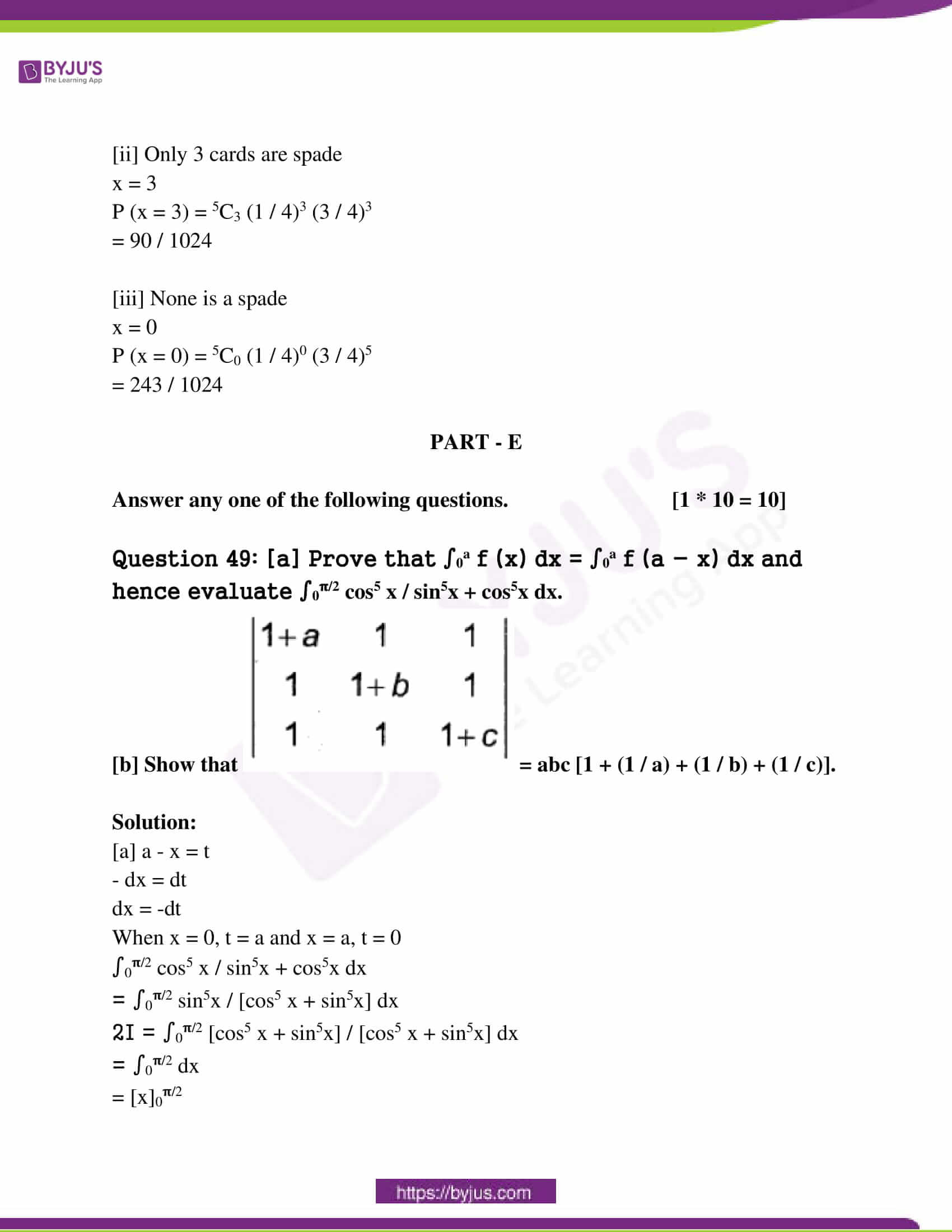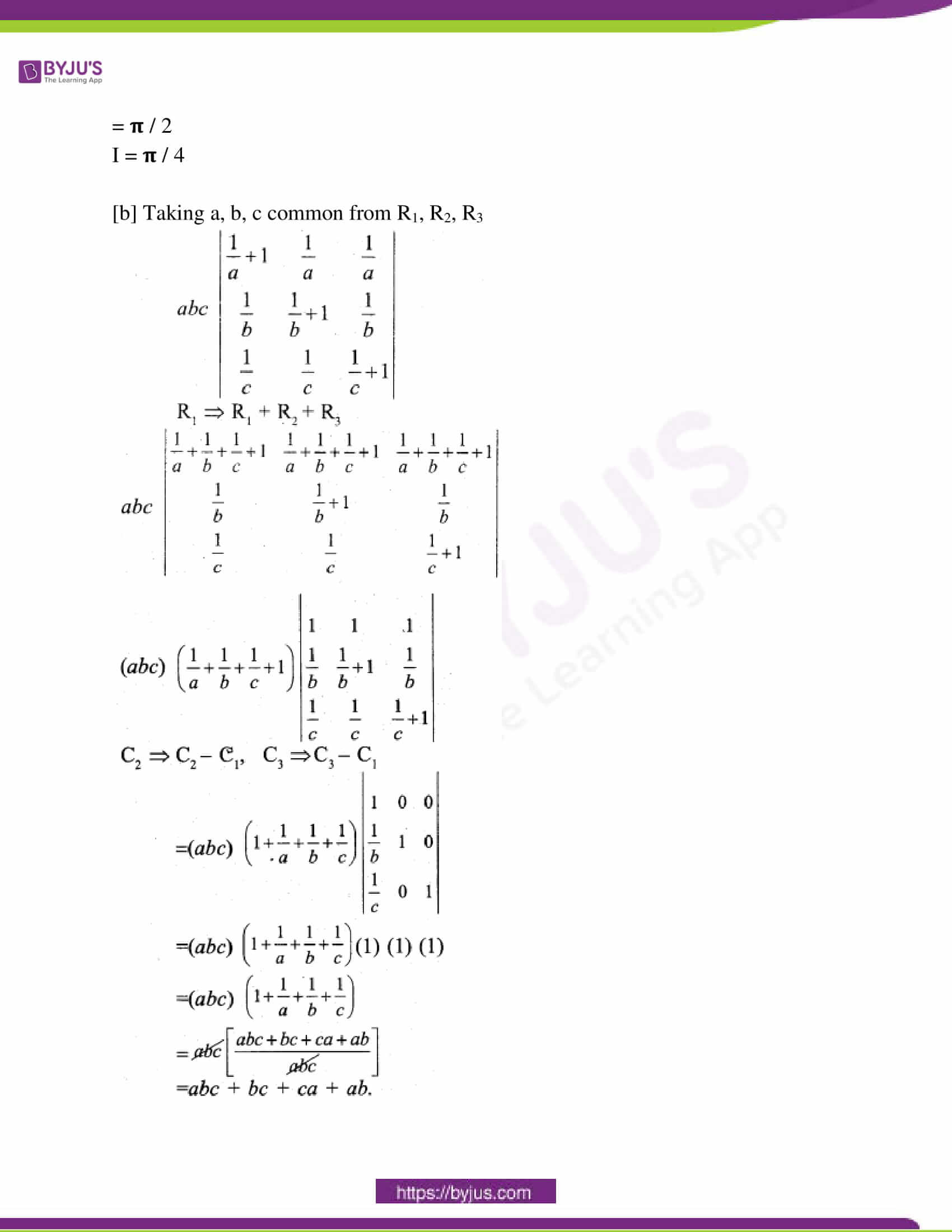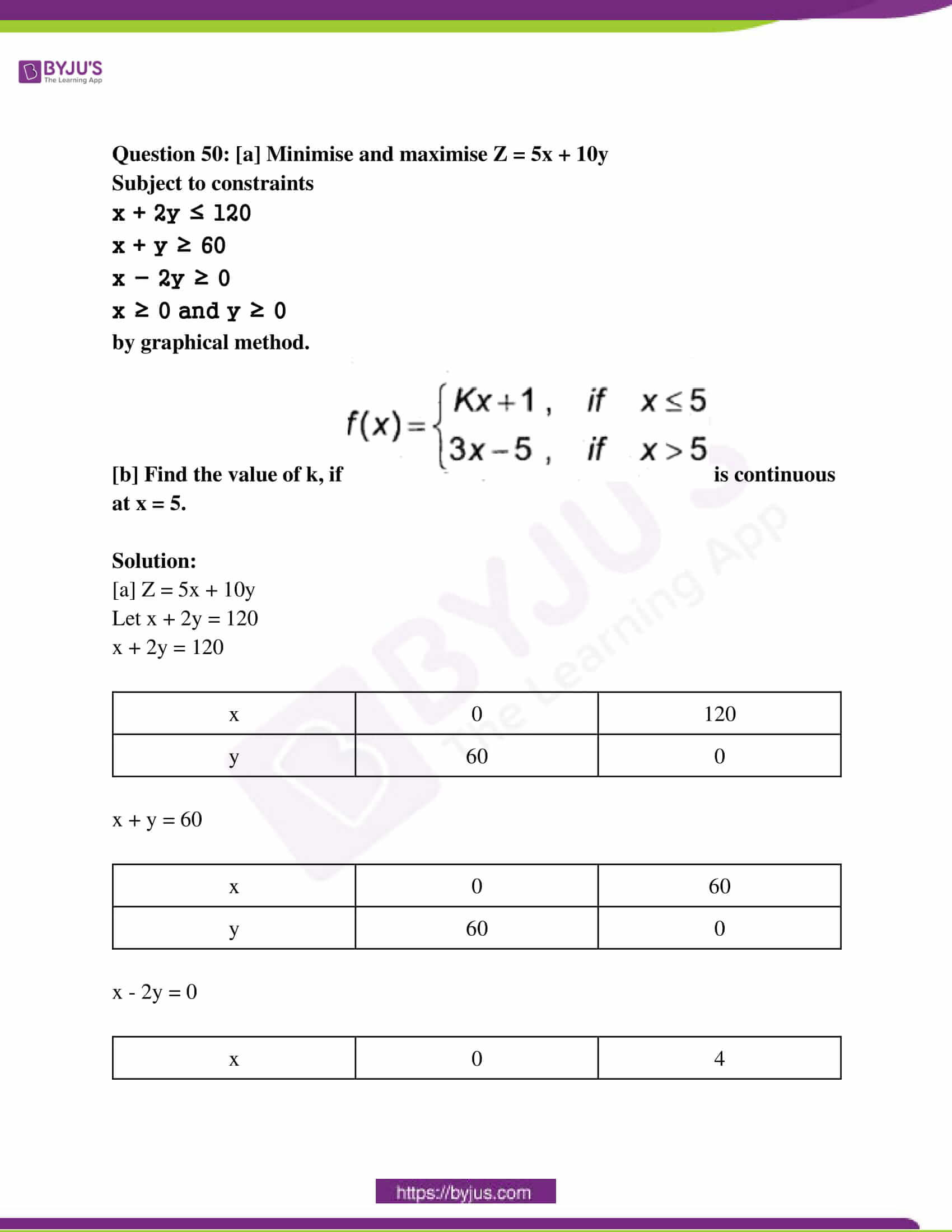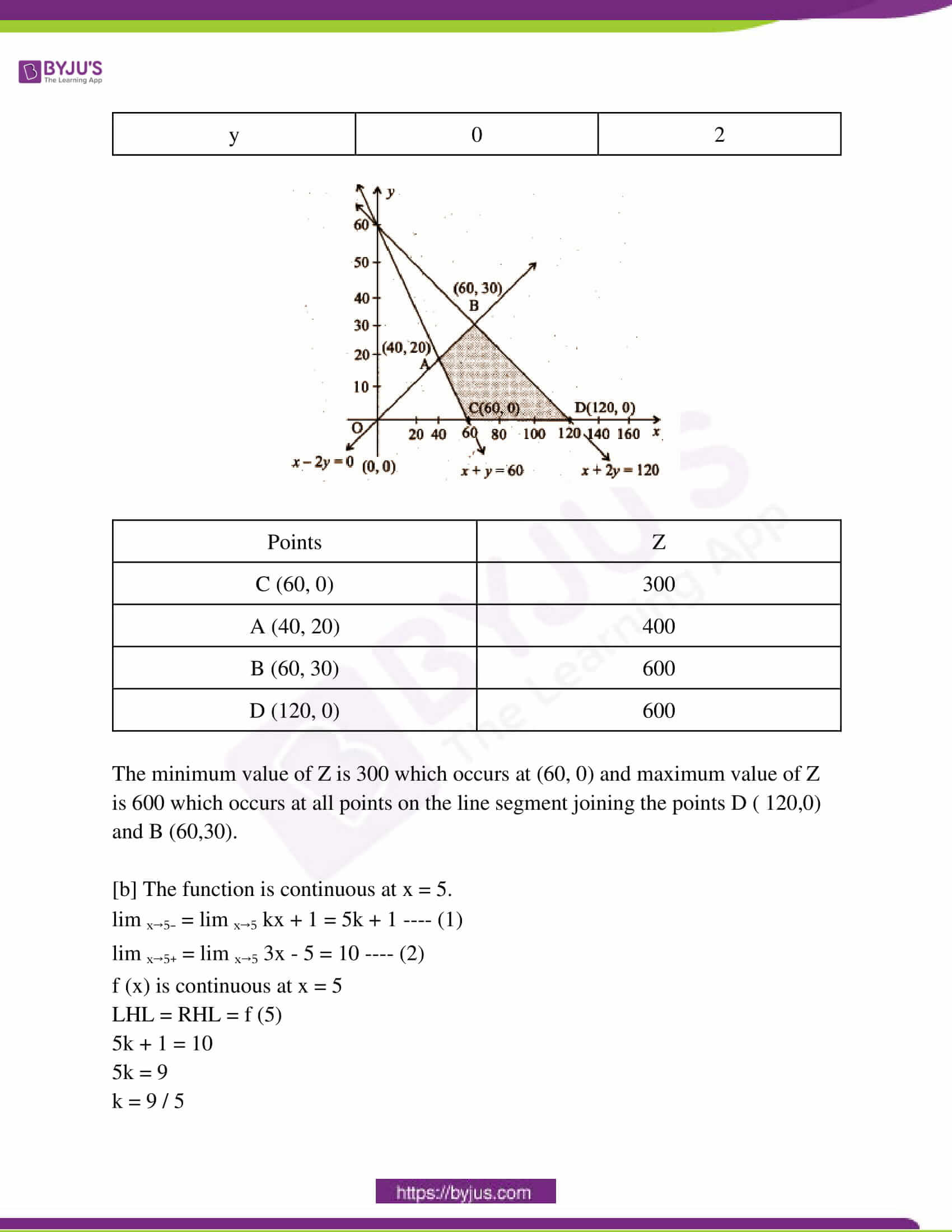PART – A

Answer all the ten questions. [10 * 1 = 10]

Question 1: Define binary operation.

Solution:

A binary operation “*” on set A is a function *: A x A → A where * is a binary operation.

Question 2: Find the principal value of cos-1 [-1 / 2].

Solution:

cos-1 [-1 / 2]

= 𝛑 – cos-1 [-1 / 2]

= 2𝛑 / 3

Question 3: Define a scalar matrix.

Solution:

A scalar matrix is a diagonal matrix in which all the diagonal elements are equal.

Question 4: Find the value of x for which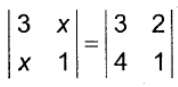.

Solution:3 – x2 = 3 – 8

3 – x2 = -5

3 + 5 = x2

x = √8 = 2√2

Question 5: If y = sin (x2 + 5) then find dy / dx.

Solution:

y = sin (x2 + 5)

dy / dx = cos (x2 + 5) * (2x)

Question 6: Find ∫(1 – x) √x dx.

Solution:

∫(1 – x) √x dx

= ∫(1 – x) x1/2 dx

= ∫x1/2 dx – ∫x3/2 dx

= (2 / 3) x3/2 – (2 / 5) x5/2 + c

Question 7: Find a value of x for which x (i + j + k) is a unit vector.

Solution:

x (i + j + k)

xi + xj + xk = 1

√x2 + x2 + x2 = 1

√3x2 = 1

x = ± 1 / √3

Question 8: If a line has direction ratios 2, -1, -2 then determine its direction cosines.

Solution:

cos ɑ = 2 / √9 = 2 / 3

cos β = -1 / √9 = -1 / 3

cos 𝝲 = -2 / √9 = -2 / 3

Question 9: Define an objective function in a linear programming problem.

Solution:

An objective function is given by Z = ax + by, where a and b are constants which can be optimised [maximised or minimised].

Question 10: If P (E) = 0.6, P (F) = 0.3, P (E ⋂ F) = 0.2, then find P (F / E).

Solution:

P (F / E) = P (E ⋂ F) / P (E)

= 0.2 / 0.6

= 0.34

PART – A

Answer any ten questions. [10 * 2 = 20]

Question 11: Show that the function f : N → N given by f (x) = 2x is one-one but not onto.

Solution:

f (x) = 2x

f (x1) = f (x2)

2x1 = 2x2

x1 = x2

So, f (x) is a one-one function.

f (x) = y

2x = y

x = y / 2 ∉ N

So, f (x) is not onto function.

Question 12: Prove that sin-1 x + cos-1 x = 𝛑 / 2, x ∈ [-1, 1].

Solution:

Let sin-1 x = θ

x = sin θ —- (1)

x = cos [(𝛑 / 2) – θ]

cos-1 x = (𝛑 / 2) – θ —- (2)

θ + cos-1 x = (𝛑 / 2)

sin-1 x + cos-1 x

= θ + (𝛑 / 2) – θ

= (𝛑 / 2)

Question 13: Write cot-1 (1 / √x2 – 1), x > 1 in the simplest form.

Solution:

cot-1 (1 / √x2 – 1)

Put x = sec θ

= cot-1 (1 / √sec2 θ – 1)

= cot-1 (1 / √tan2 θ)

= cot-1 (1 / tan θ)

= cot-1 (cot θ)

= θ

Question 14: Find the area of a triangle with vertices (2, 7), (1, 1) and (10, 8) using the determinant method.

Solution:

Area of triangle = (1 / 2)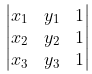= (1 / 2)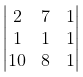= (1 / 2) [2 (1 – 8) – 7 (1 – 10) + 1 (8 – 10)]

= (1 / 2) [- 14 + 63 – 2]

= 47 / 2 square units

Question 15: Find dy / dx, if y = (log x)cosx.

Solution:

y = (log x)cosx

Applying log on both sides,

log y = cos x log (log x)

Differentiate with respect to x

(1 / y) (dy / dx) = cos x (1 / log x) * (d / dx) (logx) + log (log x) (sinx)

(1 / y) (dy / dx) = (cos x / x log x) – log (log x) (sinx)

dy / dx = (log x)cosx [(cos x / x log x) – sinx log (log x)]

Question 16: If ax + by2 = cos y then find dy / dx.

Solution:

ax + by2 = cos y

Differentiate with respect to x

a + 2by (dy / dx) = – siny (dy / dx)

dy / dx = -a / (2by + siny)

Question 17: Find the approximate change in volume V of a cube of side x meters caused by increasing side by 2%.

Solution:

The volume of the cube, V = x3

𝛿V = 3x2 * 0.02x = 0.06 x3m3

dV / dx = 3x2

dV = 3x2 * 𝛿x

= 3x2 * (0.02x)

= 0.06 x3m

Question 18: Find ∫[1 / cos2 x (1 – tan x)2] dx.

Solution:

∫[1 / cos2 x (1 – tan x)2] dx

Put 1 – tan x = t

– sec2 x dx = dt

– dt = dx / cos2 x

I = ∫[1 / cos2 x (1 – tan x)2] dx

= ∫(1 / t2) [- dt]

= ∫-t-2 dt

= -t-1 / -1 + c

= 1 / t + c

= 1 / (1 – tan x) + c

Question 19: Find ∫sin 2x * cos 3x dx

Solution:

sin x cos y = (1 / 2) sin (x + y) + sin (x – y)

∫sin 2x * cos 3x dx — (1)

= (1 / 2) [sin 5x + sin (-x)]

= sin 5x / 2 – sinx / 2

(1) becomes,

= ∫[sin 5x / 2] dx – [sinx / 2] dx

= (1 / 2) [(-cos 5x / 5) + cos x]

= -cos 5x / 10 + cos x / 2 + c

Question 20: Find the order and degree of the differential equation (d2y / dx2)2 + cos (dy / dx) = 0.

Solution:

Order of the differential equation is two, and the degree is not defined.

Question 21: If (a + b) . (a – b) = 8 and |a| = 8|b|, then find |b|.

Solution:

(a + b) . (a – b) = 8

(a . a) + (a . -b) + (b . a) – (b . b) = 8

|a|2 – |b|2 = 8

(8 |b|)2 – |b|2 = 8

64|b|2 – |b|2 = 8

|b|2 = 8 / 63

|b| = 2√2 / 3√7

Question 22: Find the projection of the vector a = 2i + 3j + 2k on the vector b = i + 2j + k.

Solution:

a = 2i + 3j + 2k

b = i + 2j + k

Projection of a on b = (a . b) / |b|

|b| = √1 + 4 + 1 = √6

= (2i + 3j + 2k) . (i + 2j + k) / √6

= (2 + 6 + 2) / √6

= 10 / √6

Question 23: Find the distance of the point (3, -2, 1) from the plane 2x – y + 2z + 3.

Solution:

The distance of the point (3, -2, 1) from the plane 2x – y + 2z + 3 is given by

p = |[(ax1 + by1 + cz1) / √a2 + b2 + c2]|

= |[(2 * 3 – (-2) + 2 (1) + 3) / (√4 + 1 + 4)]|

= 13 / 3

Question 24: Probability of solving the specific problem independently by A and B are ½ and ⅓, respectively. Suppose both try to solve the problem independently, find the probability that the problem is solved.

Solution:

P (A) = 1 / 2

P (B) = 1 / 3

P (A ⋃ B) = P (A) + P (B) – P (A ⋂ B)

A and B are independent events,

P (A ⋂ B) = P (A) * P (B)

P (A ⋃ B) = P (A) + P (B) – P (A) * P (B)

= (1 / 2) + (1 / 3) – (1 / 2) (1 / 3)

= (5 / 6) – (1 / 6)

= 4 / 6

= 2 / 3

PART – C

Answer any ten questions. [10 * 3 = 30]

Question 25: Check whether the relation R in R of the real numbers defined by R = {(a, b) : a ≤ b3} is reflexive, symmetric or transitive.

Solution:

A relation is said to be a reflexive relation if every element of A is related to itself.

Thus, (a, a) ∈ R, ∀ a ∈ A

=> R is reflexive

A relation is said to be symmetric relation, if

(a, b) ∈ R => (b, a) ∈ R , ∀ a, b ∈ A

e.g., aRb => bRa, for all a, b ∈ A

=> R is symmetric.

A relation is said to be transitive relation if

(a, b) ∈ R and (b, c) ∈ R => (a,c) ∈ R ∀ a,b,c ∈ A

R = {(a,b) : a ≤ b³ }

(1 / 3, 1 / 3) ∉ R as ⅓ ≤ ⅓2

R is not reflexive.

(1, 3) ϵ R (as 1 < 3³ = 27)

But, (3, 1) ∉ R (as 3³ > 1)

R is not symmetric.

(3, 3 / 2) , (3 / 2, 6 / 5) ∈ R

3 < (3 / 2)³ and (3 / 2) < (6 / 5)³

but (3, 6 / 5) ∉ R as 3 > (6 / 5)³

R is not transitive.

Question 26: Prove that cos-1 (4 / 5) + cos-1 (12 / 13) = cos-1 (33 / 65).

Solution:

cos-1 (4 / 5) + cos-1 (12 / 13)

cos-1 (4 / 5)

cos A = 4 / 5

sin A = √1 – (16 / 25)

= √9 / 25

= 3 / 5

cos-1 (12 / 13)

cos B = 12 / 13

sin B = √1 – (144 / 169)

= √25 / 169

= 5 / 13

cos (a + b) = cos a cos b – sin a sin b

= (4 / 5) * (12 / 13) – (3 / 5) * (5 / 13)

= (48 – 15) / 65

cos (a + b) = 33 / 65

cos (cos-1 (4 / 5) + cos-1 (12 / 13)) = (33 / 65)

cos-1 (4 / 5) + cos-1 (12 / 13) = cos-1 (33 / 65)

Question 27: By using elementary operations, find the inverse of the matrix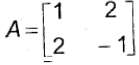.

Solution: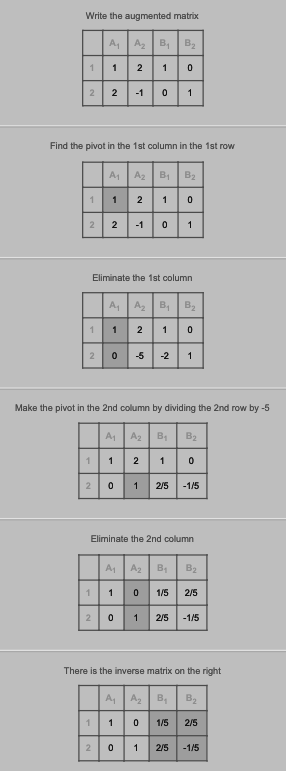Question 28: If x = a (θ + sin θ), y = a (1 – cos θ) then show that dy / dx = tan (θ / 2).

Solution:

x = a (θ + sin θ)

dx / dθ = a (1 + cos θ)

y = a (1 – cos θ)

dy / dθ = a sin θ

dy / dx = (dy / dθ) / (dx / dθ)

= a sin θ / a (1 + cos θ)

= sin θ / (1 + cos θ)

= [2 sin (θ / 2) cos (θ / 2)] / [2 cos2 (θ / 2)]

= sin (θ / 2) / cos (θ / 2)

= tan (θ / 2)

Question 29: Verify Rolle’s theorem for the function f (x) = x2 + 2x – 8, x ∈ [- 4, 2].

Solution:

Since the given function is a polynomial function, it is continuous at [- 4, 2].

f ‘ (x) = 2x + 2

The given function is differentiable at [- 4, 2].

f (- 4) = 16 – 8 – 8 = 0

f (2) = 4 + 4 – 8 = 0

f (- 4) = f (2) at x ∈ [- 4, 2]

By Rolles’ theorem, there exists a real valued function c ∈ [- 4, 2]

f ‘ (c) = 0

2c + 2 = 0

2c = – 2

c = -1 ∈ [- 4, 2]

Thus Rolle’s theorem is verified.

Question 30: Find the intervals in which the function f given by f (x) = 2x3 – 3x2 – 36x + 7 is strictly increasing.

Solution:

f (x) = 2x3 – 3x2 – 36x + 7

f ‘ (x) > 0

f ‘ (x) = 6x2 – 6x – 36

[6x2 – 6x – 36] > 0

6 [x2 – x – 6] > 0

x2 – 3x + 2x – 6 = 0

x (x – 3) + 2 (x – 3) = 0

(x + 2) (x – 3) = 0

x = 3, -2

f (x) is strictly increasing at (-2, 3).

Question 31: Find ∫x log x dx.

Solution:

∫x log x dx

By using integrating by parts,

= log x ∫x dx – ∫(d [log x] / dx) * [∫x dx] dx

= log x * (x2 / 2) – ∫(1 / x) * (x2 / 2) dx

= log x * (x2 / 2) – (1 / 2) (x2 / 2) + c

Question 32: Evaluate ∫0𝛑 / 2 sinx / (1 + cos2 x) dx.

Solution:

I = ∫0𝛑 / 2 sinx / (1 + cos2 x) dx

= ∫0𝛑 / 2 – d (cosx) / (1 + cos2 x)

= ∫0𝛑 / 2 d (cosx) / (1 + cos2 x)

= [- tan-1 (cosx)]0𝛑 / 2

= – tan-1 (cos [𝛑 / 2]) + tan-1 (cos 0)

= 0 + 𝛑 / 4

= 𝛑 / 4

Question 33: Find the area of the region bounded by the curve y2 = 4x and the line x = 3.

Solution:

Required area = 2 ∫03 2√x dx

= 2 * 2 ∫03 x1/2 dx

= 4 * (2 / 3) [(x)3/2]03

= (8 / 3) * [(3)3/2 – (0)3/2]

= (8 / 3) √27

= 8√3 square units

Question 34: Form the differential equation of the family of curves y = ae3x + be-2x by eliminating arbitrary constants a and b.

Solution:

y = ae3x + be-2x

dy / dx = 3ae3x – 2be-2x

d2y / dx2 = 9ae3x + 4be-2x

y = ae3x + be-2x

(y – be-2x) / a = e3x

dy / dx = 3a * [(y – be-2x) / a] – 2be-2x

= 3y – 3be-2x – 2be-2x

= 3y – 5be-2x

Question 35: Find a unit vector perpendicular to each of the vectors (a + b) and (a – b), where a = i + j + k, b = i + 2j + 3k.

Solution:

a = i + j + k

b = i + 2j + 3k

a + b = 2i + 3j + 4k

a – b = 0i – j – 2k

(a + b) x (a – b)

=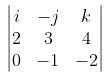= i (- 6 + 4) + j (- 4 – 0) + k (- 2 – 0)

c = – 2i – 4j – 2k

c = c / |c|

= [- 2i – 4j – 2k] / √4 + 16 + 4

= [- 2i – 4j – 2k] / √24

Question 36: Show that the four points with position vectors are 4i + 8j + 12k, 2i + 4j + 6k, 3i + 5j + 4k and 5i + 8j + 5k are coplanar.

Solution:

OA = 4i + 8j + 12k

OB = 2i + 4j + 6k

OC = 3i + 5j + 4k

OD = 5i + 8j + 5k

AB = OB – OA = – 2i – 4j – 6k

AC = OC – OA = – i – 3j – 8k

AD = OD – OA = i + 0j – 7k

The condition to be coplanar =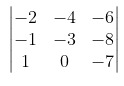= – 2 [21 + 0] + 4 [7 + 8] – 6 [0 + 3]

= – 42 + 60 – 18

= 0

So, the points are coplanar.

Question 37: Find the vector equation of the plane passing through the points R (2, 5, -3), S (-2, -3, 5) and T (5, 3, -3).

Solution:

Let a = 2i + 5j – 3k

b = -2i – 3j + 5k

c = 5i + 3j – 3k

The vector equation is (r – a) [(b – a) x (c – a)] = 0

The intercept form of the equation of a plane.

Let the equation of a plane be Ax + By + Cz + 0 = 0 —– (1)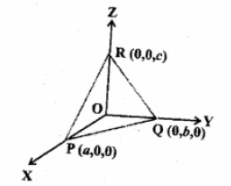Let the plane cut the x-axis at p (a, 0, 0), y-axis at Q (0, b, 0), z-axis at R (0, 0, c).

Substituting P (a, 0, 0) in equation (1),

Aa + D = 0

⇒ Aa = – D

⇒ A = -D / a

Substituting Q (0, b, 0) in equation (1),

Bb + D = 0

⇒ Bb = D

⇒ B = -D / b

Similarly R(0, 0, c) in equation (1),

Cc + D = 0

⇒ Cc = -D

⇒ C = -D / c

Substituting A, B, C in (1),

(-D / a) x + (-D / b) y + (-D / c) z = -D

Divide theroughtout by -D,

(x / a) + (y / b) + (z / c) = 1 is the required equation of the plane in intercept form.

Question 38: An insurance company insured 2000 scooter drivers, 4000 car drivers and 6000 truck drivers. The probability of an accident is 0.01, 0.03 and 0.15, respectively. One of the insured persons meets with an accident. What is the probability that he is a scooter driver?

Solution:

Total number of vehicles = 2000 + 4000 + 6000 = 12000

Let

E1 = event occurred by a scooter driver

P (E1) = 2000 / 12000 = 1 / 6

E2 = event occurred by a car driver

P (E2) = 4000 / 12000 = 1 / 3

E3 = event occurred by a truck driver

P (E3) = 6000 / 12000 = 1 / 2

P (A / E1) = 0.01 = 1 / 100

P (A / E2) = 0.03 = 3 / 100

P (A / E3) = 0.15 = 15 / 100

Baye’s theorem can be applied.

P (E1 / A) = [{P (E1) * P (A / E1)} / {{P (E1) * P (A / E1) + {P (E2) * P (A / E2) + {P (E3) * P (A / E3)}]

= [{(1 / 6) * (1 / 100)} / {(1 / 6) * (1 / 100) + {(1 / 3) * (3 / 100) + {(1 / 2) * (15 / 100)]

= [{1 / 6} / [(1 / 6) + 1 + (15 / 2)]

= 1 / 52

PART – D

Answer any six questions. [6 * 5 = 30]

Question 39: Let f : N → Y be a function defined as f (x) = 4x + 3, where y = {y ∈ N : y = 4x + 3 for some x ∈ N}. Show that f is invertible. Find the inverse of f.

Solution:

One to one function: f (x1) = f (x2)

4x1 + 3 = 4x2 + 3

4x1 = 4x2

x1 = x2 ∀ x ∈ N

f is one-one function

Onto: y = f (x)

= 4x + 3

4x = y – 3

x = (y – 3) / 4 ∀ x ∈ N, y ∈ N

Hence, f is onto function.

f is invertible ∀ x ∈ N, y ∈ N.

f-1 = (y – 3) / 4

Question 40: If A =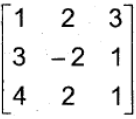then show that A3 – 23A – 40I = 0.

Solution: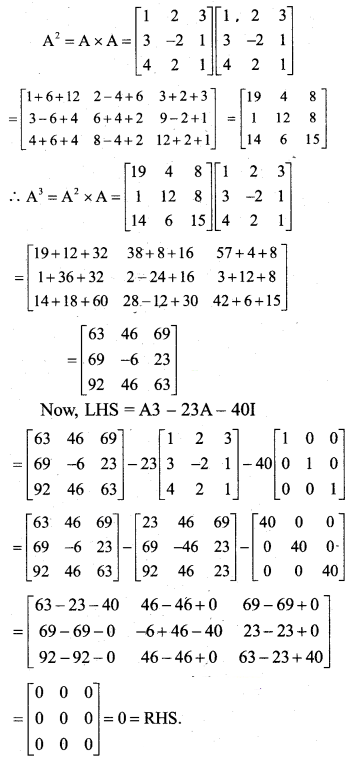Question 41: Solve the following system of linear equations by matrix method.

3x – 2y + 3z = 8

2x + y – z = 1

4x – 3y + 2z = 4

Solution:

A . X = B

X = A-1 B

|A| = det A =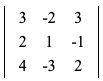= 3·1·2 + (-2)·(-1)·4 + 3·2·(-3) – 3·1·4 – 3·(-1)·(-3) – (-2)·2·2 = 6 + 8 – 18 – 12 – 9 + 8

= -17

Matrix of cofactors =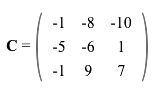Transposed matrix of cofactors =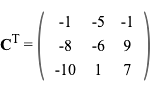Inverse = Transpose matrix of cofactors / |A| =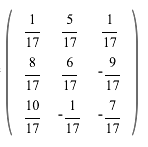X = A-1 B =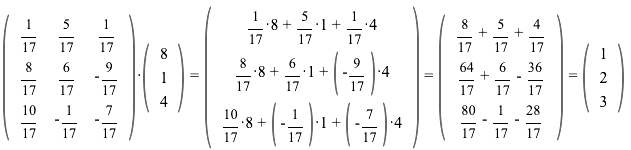x = 1, y = 2, z = 3

Question 42: If y = sin-1 x, show that (1 – x2) (d2y / dx2) – x (dy / dx) = 0.

Solution:

y = sin-1 x

Differentiate with respect to x,

dy / dx = 1 / √1 – x2

(√1 – x2) (dy / dx) = 1

Again differentiating with respect to x,

(√1 – x2) (d2y / dx2) + (dy / dx) (1 / 2 √1 – x2) * (d / dx) (1 – x2)

(√1 – x2) (d2y / dx2) + (dy / dx) (-2x / 2√1 – x2) = 0

(√1 – x2) (d2y / dx2) = (dy / dx) (x / √1 – x2)

(√1 – x2) (d2y / dx2) = (dy / dx) x

(1 – x2) (d2y / dx2) = x (dy / dx)

(1 – x2) (d2y / dx2) – x (dy / dx) = 0

Question 43: The length x of a rectangle is decreasing at the rate of 3 cm/min and the width y is increasing at the of 2 cm/min. When x = 10 cm and y = 6cm, find the rates of change of

i) The perimeter and

ii) The area of the rectangle.

Solution:

[i] Let x and y be the length and breadth of the rectangle.

dx / dt = 3 cm/min

dy / dt = 2 cm/min

x = 10cm y = 6 cm

Perimeter of the rectangle = 2 (l + b)

P = 2 (x + y)

dP / dt = 2 [dx / dt + dy / dt]

= 2 [-3 + 2]

= -2 cm/min

The perimeter of the rectangle is decreasing at the rate of 2cm/min.

[ii] The area of the rectangle = length * breadth

A = xy

dA / dt = x * (dy / dt) + y * (dx / dt)

= 10 * 2 + 6 * (- 3)

= 20 – 18

= 2cm2/min

The area of the rectangle is increasing at the rate of 2cm2/min.

Question 44: Find the integral of 1 / x2 – a2 with respect to x and hence evaluate ∫1 / x2 – 16 dx.

Solution:

I = ∫[1 / x2 – a2] dx

= ∫[(1 / x – a) * (1 / x + a)] dx

= [2a / 2a] ∫[(1 / x – a) * (1 / x + a)] dx

= (1 / 2a) ∫[2a / (x – a) (x + a)] dx

= (1 / 2a) ∫[(x + a) – (x – a) / (x + a) (x – a)] dx

= (1 / 2a) ∫[(1 / x – a) – (1 / x + a)] dx

= (1 / 2a) [∫(1 / x – a) dx – ∫(1 / x + a) dx]

= (1 / 2a) [log (x – a) – log (x + a)] + c

= (1 / 2a) log [|x – a| / |x + a|] + c

∫1 / x2 – 16 dx

= ∫1 / x2 – 42 dx

By (1 / 2a) log [|x – a| / |x + a|] + c

= (1 / 8) log |(x – 4) / (x + 4)| + c

Question 45: Using the method of integration, find the smaller area enclosed by the smaller circle x2 + y2 = 4 and the line x + y = 2.

Solution:

The given circle is x2 + y2 = 4 and the line is x + y = 2.

x2 + (2 – x)2 = 4

x2 + 22 – 4x + x2 – 4 = 0

2x2 – 4x = 0

2x (x – 2) = 0

x = 0, 2

x2 + y2 = 4 and x + y = 2

y2 = 4 – x2

y = √22 – x2

Required area = A = ∫02 (√22 – x2) dx – ∫02 (2 – x) dx

= [(x / 2) (√22 – x2) + 2 sin-1 (x / 2)]02 – 2 (x)02 + [x2 / 2]02

= [(0 + 2 sin-1 (1)) – 0] – 2 [2 – 0] + (2 – 0)

= 2 (𝛑 / 2) – 4 + 2

= [𝛑 – 2] square units

Question 46: Find the general solution of the differential equation dy / dx + (secx) y = tanx, (0 ≤ x ≤ 𝛑 / 2).

Solution:

dy / dx + (secx) y = tanx

dy / dx + Py = Q

P = secx, Q = tanx

IF = e∫P dx

= e∫secx dx

= elog (secx + tanx)

= secx + tanx + c

The general solution is IF * y = ∫Q * IF dx + c

(secx + tanx) y = ∫(secx + tanx) secx dx

(secx + tanx) y = ∫sec2 x dx + ∫secx tanx dx

(secx + tanx) y = tanx + secx + c1

y = (tanx + secx) / (tanx + secx) + c1 / tanx + secx

y = 1 + c [where c = (c1 / tanx + secx)]

Question 47: Derive the equation of a line in space passing through a given point and parallel to a given vector in both vector and cartesian form.

Solution:

Let r be any position vector on the line L and a is a given point on the line L.

Clearly AP is parallel to b.

AP = ƛb [ƛ is some real number]

AP = OA – OA

AP = r – a

r – a = ƛb

(r – a) + ƛb — (1)

Cartesian form:

Let r = xi + yj + 2k

a = x1i + y1j + 2k

b = ai + bj + ck

r – a = (x – x1) i + (y – y1) j + (z – z1) k

From (1), r – a = ƛb

(x – x1) i + (y – y1) j + (z – z1) k = ƛ [ai + bj + ck]

Equating the corresponding components of i, j, k respectively,

x – x1 = aƛ, y – y1 = bƛ, z – z1 = cƛ

ƛ = (x – x1) / a, ƛ = (y – y1) / b, ƛ = (z – z1) / c

(x – x1) / a = (y – y1) / b = (z – z1) / c

Question 48: Five cards are drawn successively with replacement from a well-shuffled deck of 52 cards. What is the probability that

i) All the five cards are spades?

ii) Only three cards are spades?

Solution:

P = (number of spade cards) / total number of cards = 13 / 52 = 1 / 4

q = 1 – p

= 1 – (1 / 4)

= 3 / 4

n = 5

P (X = x) = nCx px qn-x

= 5C2 (1 / 4)x (3 / 4)5-x

[i] For all 5 cards to be spade

x = 5

P (x = 5) = 5C5 (1 / 4)5 (3 / 4)0

= 1 / 1024

[ii] Only 3 cards are spade

x = 3

P (x = 3) = 5C3 (1 / 4)3 (3 / 4)3

= 90 / 1024

x = 0

P (x = 0) = 5C0 (1 / 4)0 (3 / 4)5

= 243 / 1024

PART – E

Answer any one of the following questions. [1 * 10 = 10]

Question 49: [a] Prove that ∫0a f (x) dx = ∫0a f (a – x) dx and hence evaluate ∫0𝛑/2 cos5 x / sin5x + cos5x dx.

[b] Show that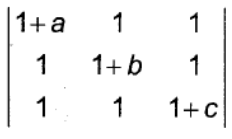= abc [1 + (1 / a) + (1 / b) + (1 / c)].

Solution:

[a] a – x = t

– dx = dt

dx = -dt

When x = 0, t = a and x = a, t = 0

0𝛑/2 cos5 x / sin5x + cos5x dx

= ∫0𝛑/2 sin5x / [cos5 x + sin5x] dx

2I = ∫0𝛑/2 [cos5 x + sin5x] / [cos5 x + sin5x] dx

= ∫0𝛑/2 dx

= [x]0𝛑/2

= 𝛑 / 2

I = 𝛑 / 4

[b] Taking a, b, c common from R1, R2, R3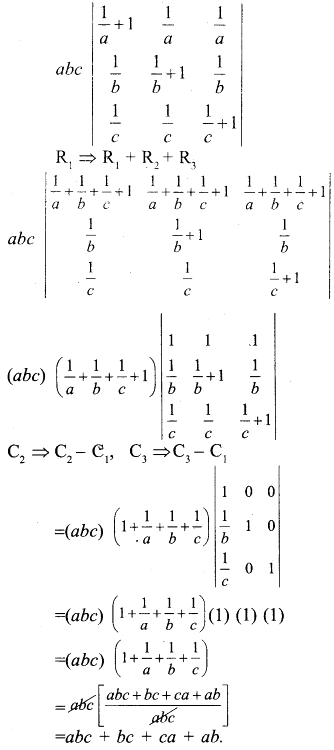Question 50: [a] Minimise and maximise Z = 5x + 10y

Subject to constraints

x + 2y ≤ 120

x + y ≥ 60

x – 2y ≥ 0

x ≥ 0 and y ≥ 0

by graphical method.

[b] Find the value of k, if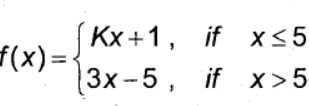is continuous at x = 5.

Solution:

[a] Z = 5x + 10y

Let x + 2y = 120

x + 2y = 120

 x 0 120 y 60 0

x + y = 60

 x 0 60 y 60 0

x – 2y = 0

 x 0 4 y 0 2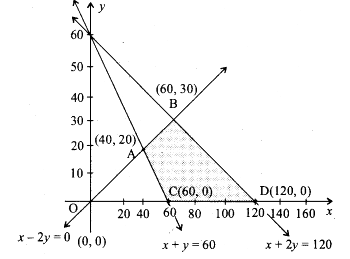Points Z C (60, 0) 300 A (40, 20) 400 B (60, 30) 600 D (120, 0) 600

The minimum value of Z is 300 which occurs at (60, 0) and maximum value of Z is 600 which occurs at all points on the line segment joining the points D ( 120,0) and B (60,30).

[b] The function is continuous at x = 5.

lim x→5- = lim x→5 kx + 1 = 5k + 1 —- (1)

lim x→5+ = lim x→5 3x – 5 = 10 —- (2)

f (x) is continuous at x = 5

LHL = RHL = f (5)

5k + 1 = 10

5k = 9

k = 9 / 5